# Mouse Visium Kidney¶

Warning

This tutorial was written with Giotto version 0.3.6.9046, your version is 1.0.3. This is a more recent version and results should be reproducible.

## Install Python and R Modules¶

To run this vignette you need to install all of the necessary Python modules.

Important

Python module installation can be done either automatically via our installation tool (from within R) (see step 2.2A) or manually (see step 2.2B).

See Part 2.2 Giotto-Specific Python Packages of our Giotto Installation section for step-by-step instructions.

## Optional: Set Giotto Instructions¶

Within R

```# to automatically save figures in save_dir set save_plot to TRUE
temp_dir = getwd()
temp_dir = '~/Temp/'
myinstructions = createGiottoInstructions(save_dir = temp_dir,
save_plot = TRUE,
show_plot = FALSE)
```

## Set-Up Giotto¶

```library(Giotto)
```

### Set A Working Directory¶

```#results_folder = '/path/to/directory/'
results_folder = '/Volumes/Ruben_Seagate/Dropbox (Personal)/Projects/GC_lab/Ruben_Dries/190225_spatial_package/Results/Visium/Brain/201226_results//'
```

### Set A Giotto Python Path¶

```# set python path to your preferred python version path
# set python path to NULL if you want to automatically install (only the 1st time) and use the giotto miniconda environment
python_path = NULL
if(is.null(python_path)) {
installGiottoEnvironment()
}
```

## Dataset Explanation¶

10X genomics recently launched a new platform to obtain spatial expression data using a Visium Spatial Gene Expression slide. The Visium brain data to run this tutorial can be found here.

High resolution png from original tissue: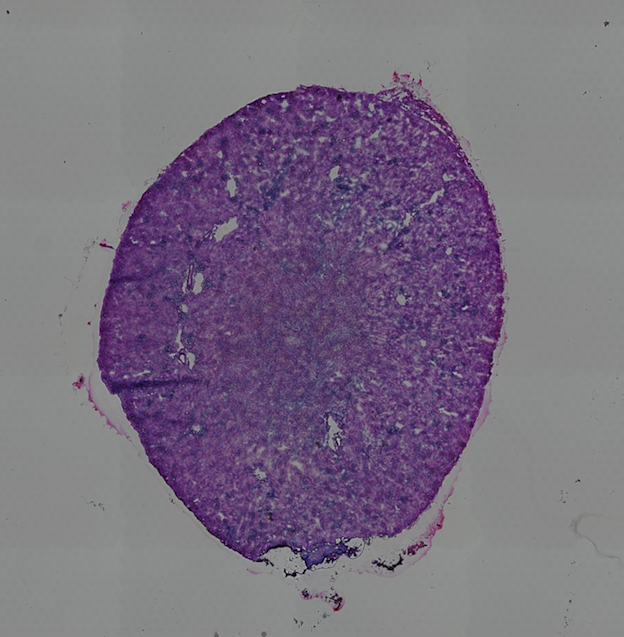## 1. Giotto Global Instructions and Preparations¶

### 1.1. Create Instructions¶

```instrs = createGiottoInstructions(save_dir = results_folder,
save_plot = TRUE,
show_plot = FALSE,
python_path = python_path)
```

### 1.2. Provide Path to Visium Folder¶

```data_path = '/path/to/Kidney_data/'
```

## 2. Create Giotto Object and Process The Data¶

```## directly from visium folder
visium_kidney = createGiottoVisiumObject(visium_dir = data_path, expr_data = 'raw',
png_name = 'tissue_lowres_image.png',
gene_column_index = 2, instructions = instrs)
```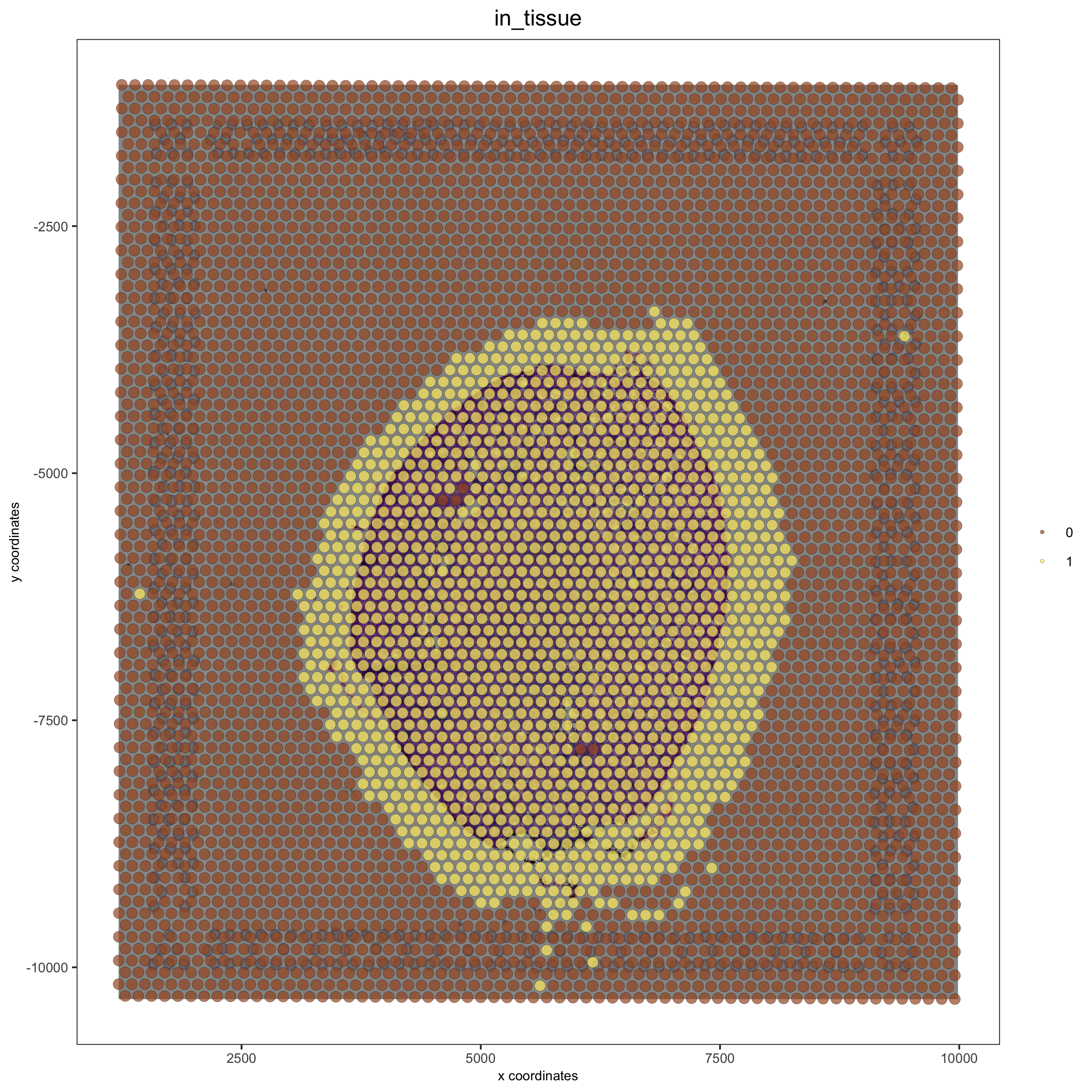```# check name
showGiottoImageNames(visium_kidney) # "image" is the default name
# adjust parameters to align image (iterative approach)
visium_kidney = updateGiottoImage(visium_kidney, image_name = 'image',

# now it's aligned
spatPlot(gobject = visium_kidney, cell_color = 'in_tissue', show_image = T, point_alpha = 0.7,
```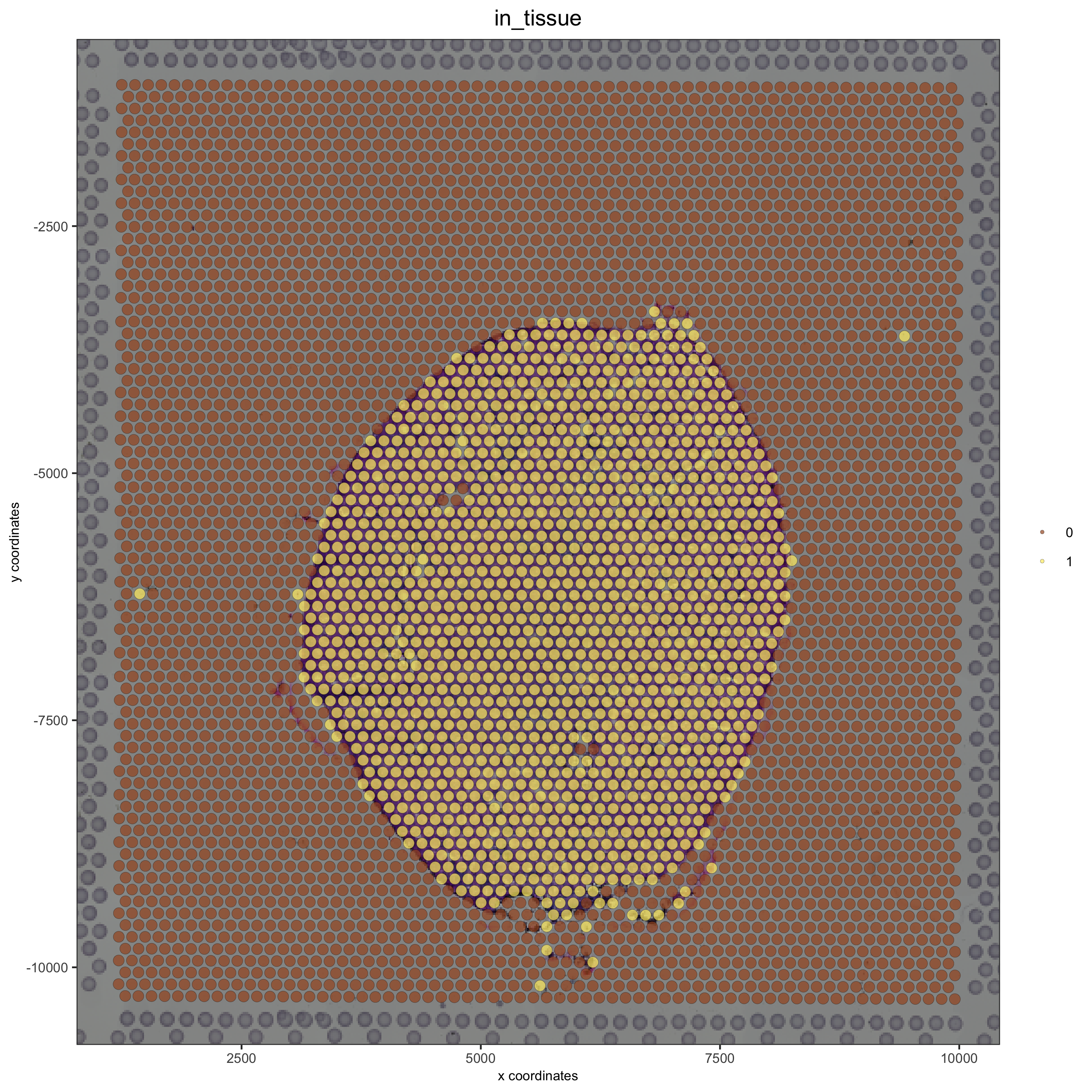```## check metadata

## compare in tissue with provided jpg
spatPlot(gobject = visium_kidney, cell_color = 'in_tissue', point_size = 2,
cell_color_code = c('0' = 'lightgrey', '1' = 'blue'),
save_param = list(save_name = '2_c_in_tissue'))
```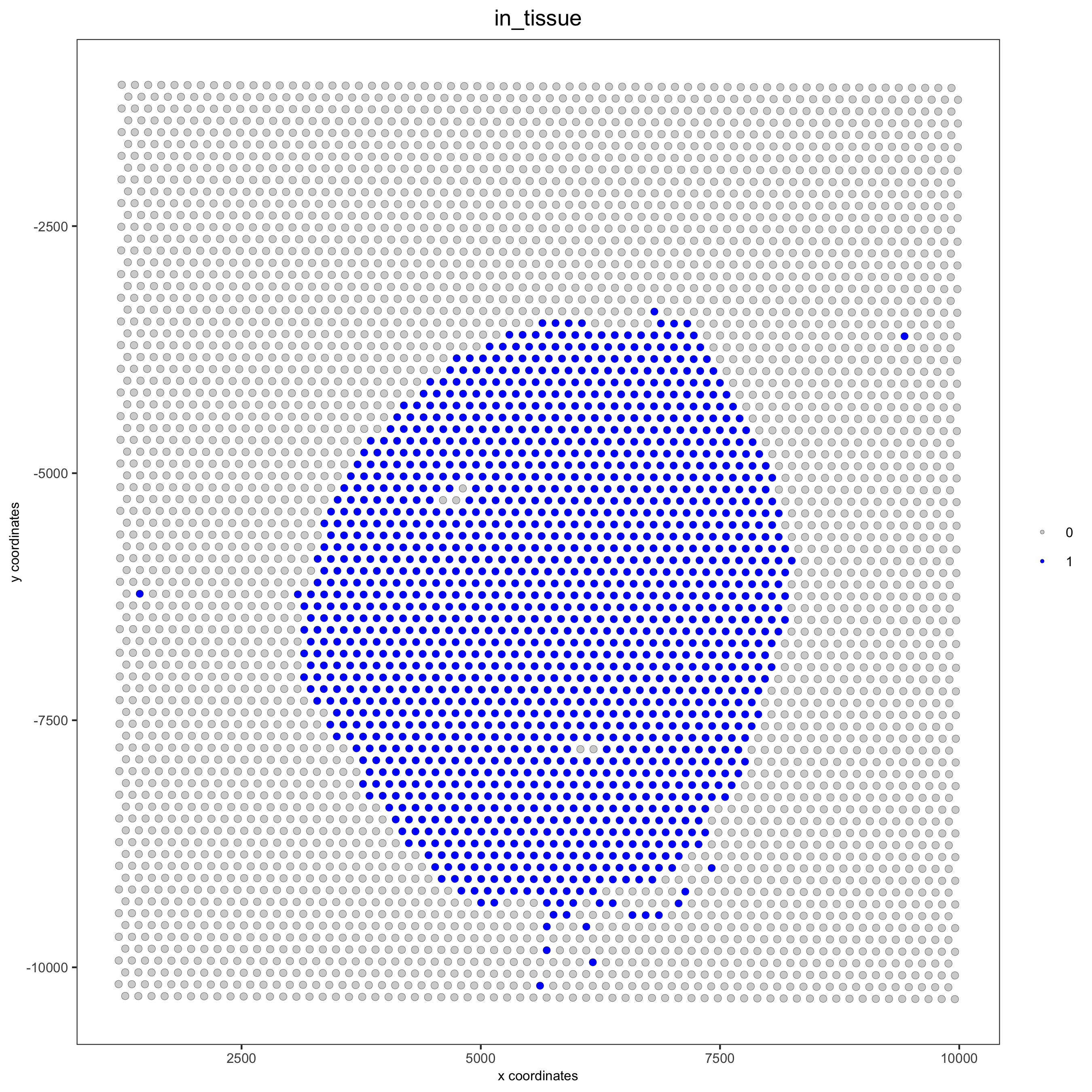```## subset on spots that were covered by tissue
visium_kidney = subsetGiotto(visium_kidney, cell_ids = in_tissue_barcodes)

## filter
visium_kidney <- filterGiotto(gobject = visium_kidney,
expression_threshold = 1,
gene_det_in_min_cells = 50,
min_det_genes_per_cell = 1000,
expression_values = c('raw'),
verbose = T)

## normalize
visium_kidney <- normalizeGiotto(gobject = visium_kidney, scalefactor = 6000, verbose = T)

## add gene & cell statistics
```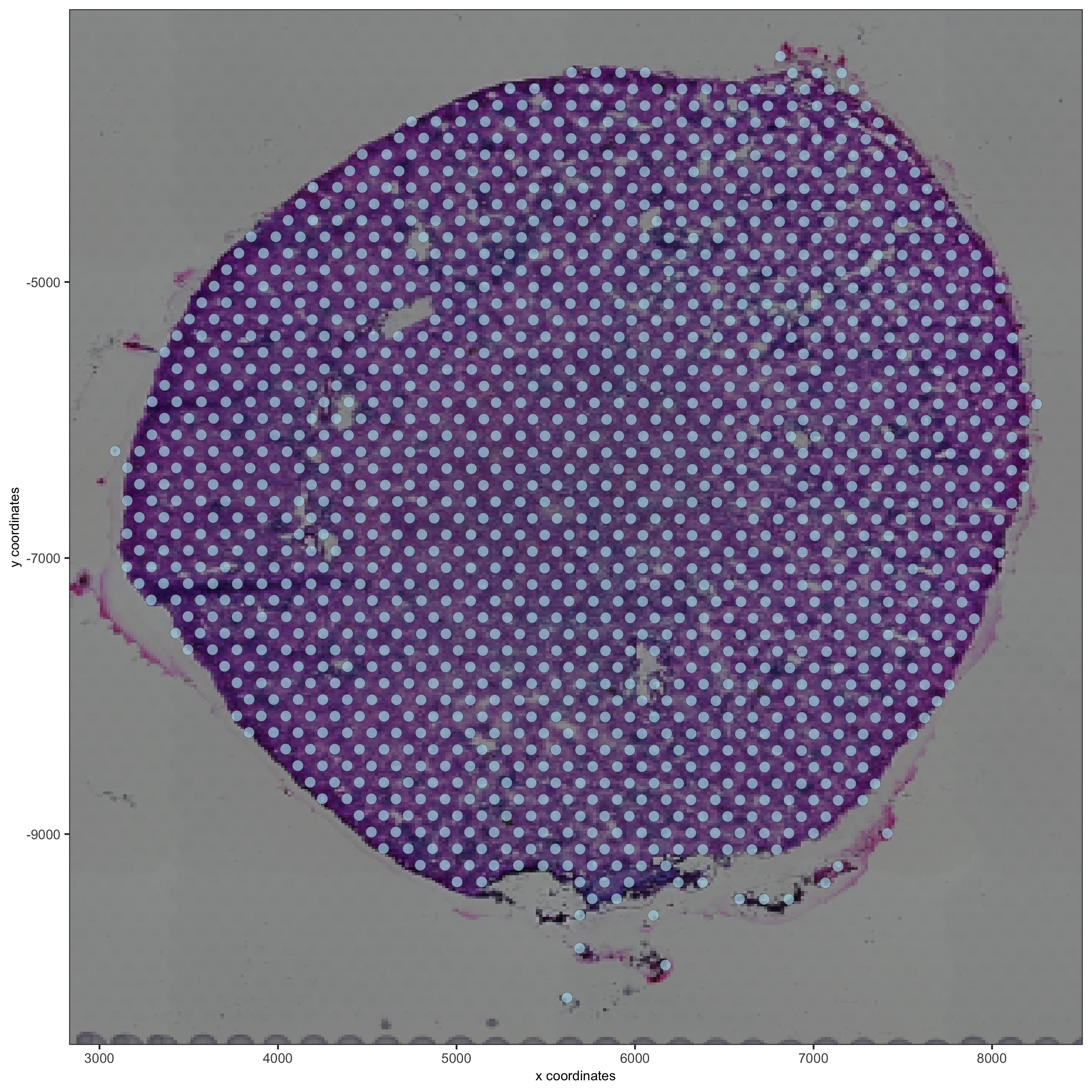```spatPlot2D(gobject = visium_kidney, show_image = T, point_alpha = 0.7,
cell_color = 'nr_genes', color_as_factor = F,
save_param = list(save_name = '2_e_nr_genes'))
```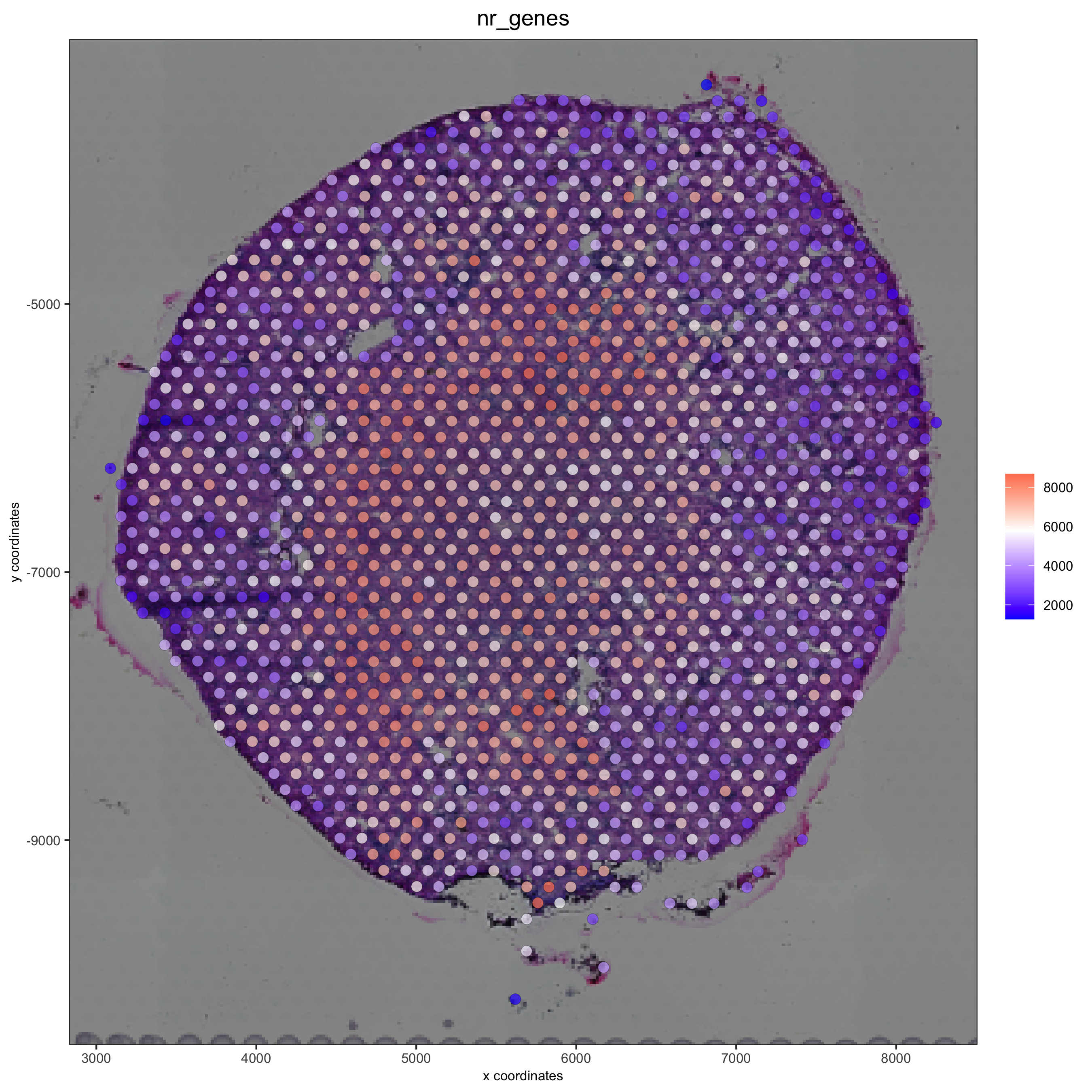## 3. Dimension Reduction¶

```## highly variable genes (HVG)
visium_kidney <- calculateHVG(gobject = visium_kidney,
save_param = list(save_name = '3_a_HVGplot'))
```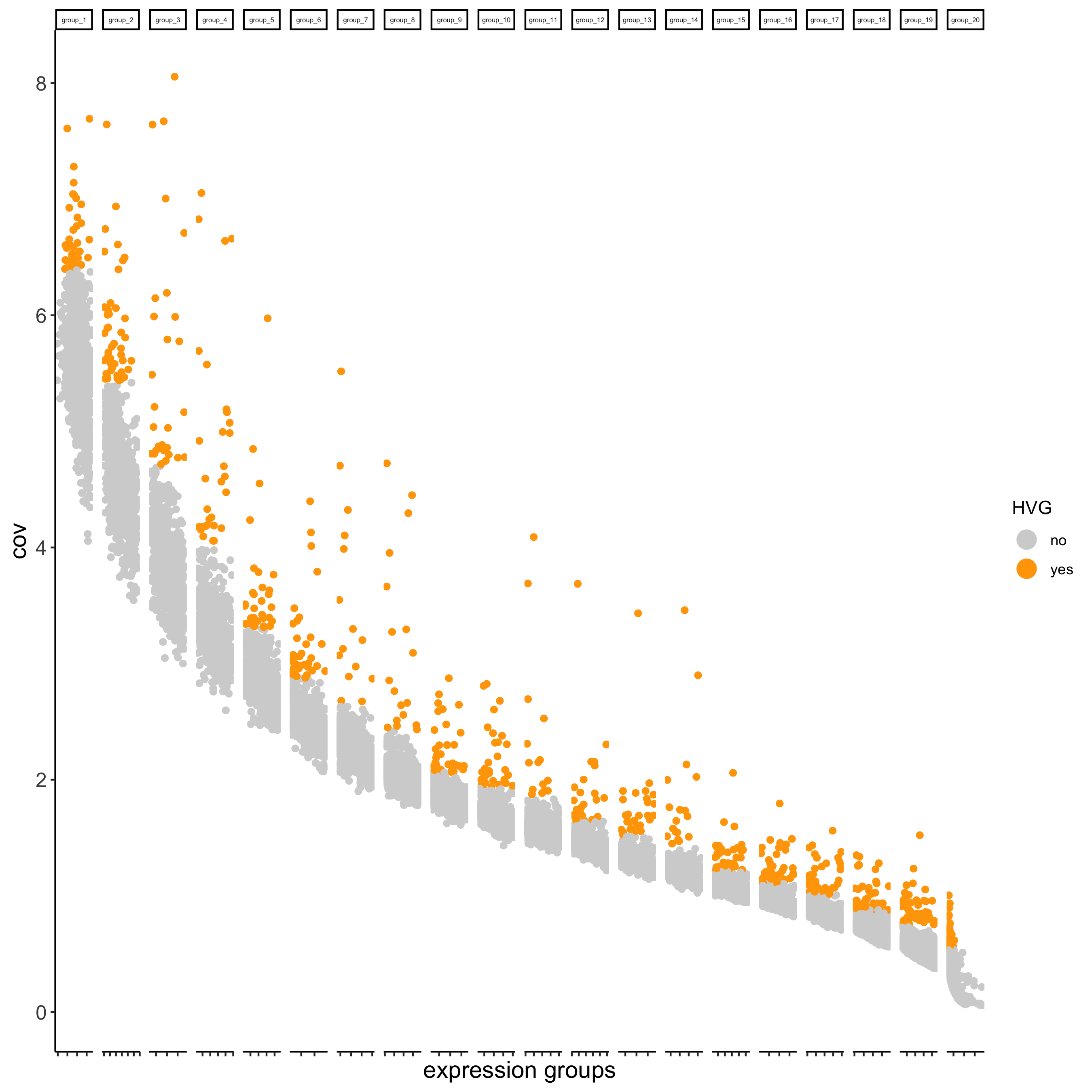```## run PCA on expression values (default)
visium_kidney <- runPCA(gobject = visium_kidney, center = TRUE, scale_unit = TRUE)
screePlot(visium_kidney, ncp = 30, save_param = list(save_name = '3_b_screeplot'))
```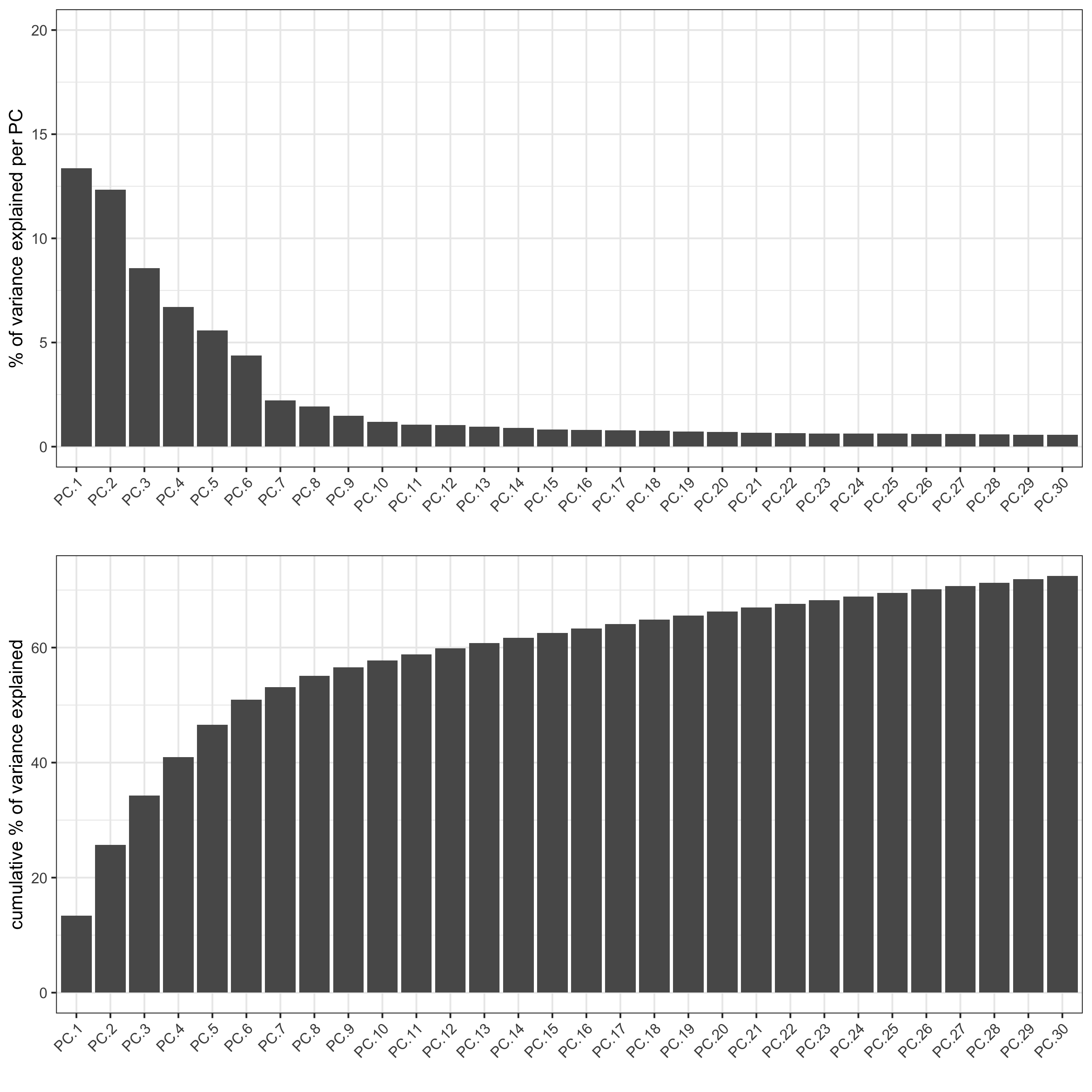```plotPCA(gobject = visium_kidney,
save_param = list(save_name = '3_c_PCA_reduction'))
```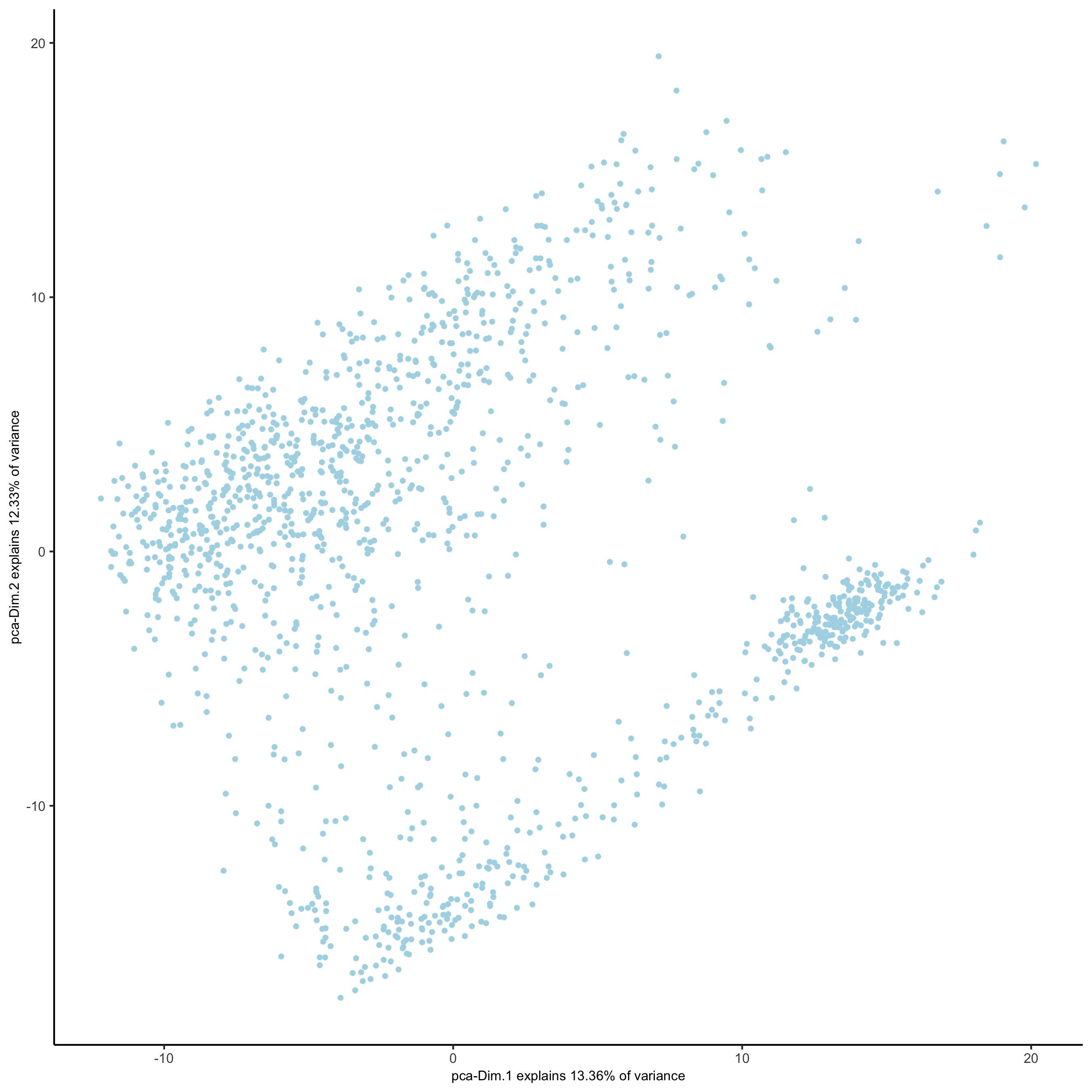```## run UMAP and tSNE on PCA space (default)
visium_kidney <- runUMAP(visium_kidney, dimensions_to_use = 1:10)
plotUMAP(gobject = visium_kidney,
save_param = list(save_name = '3_d_UMAP_reduction'))
```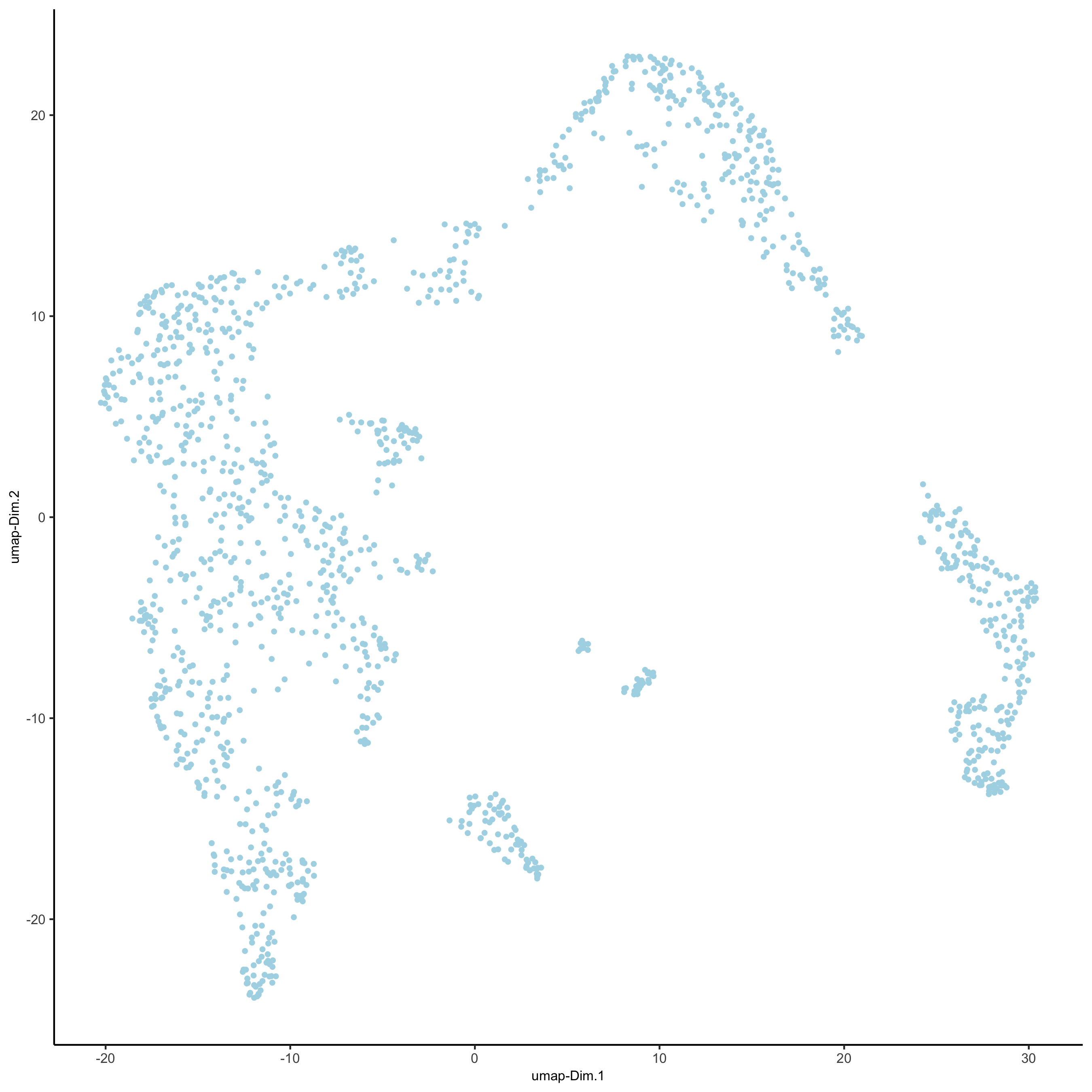```visium_kidney <- runtSNE(visium_kidney, dimensions_to_use = 1:10)
plotTSNE(gobject = visium_kidney,
save_param = list(save_name = '3_e_tSNE_reduction'))
```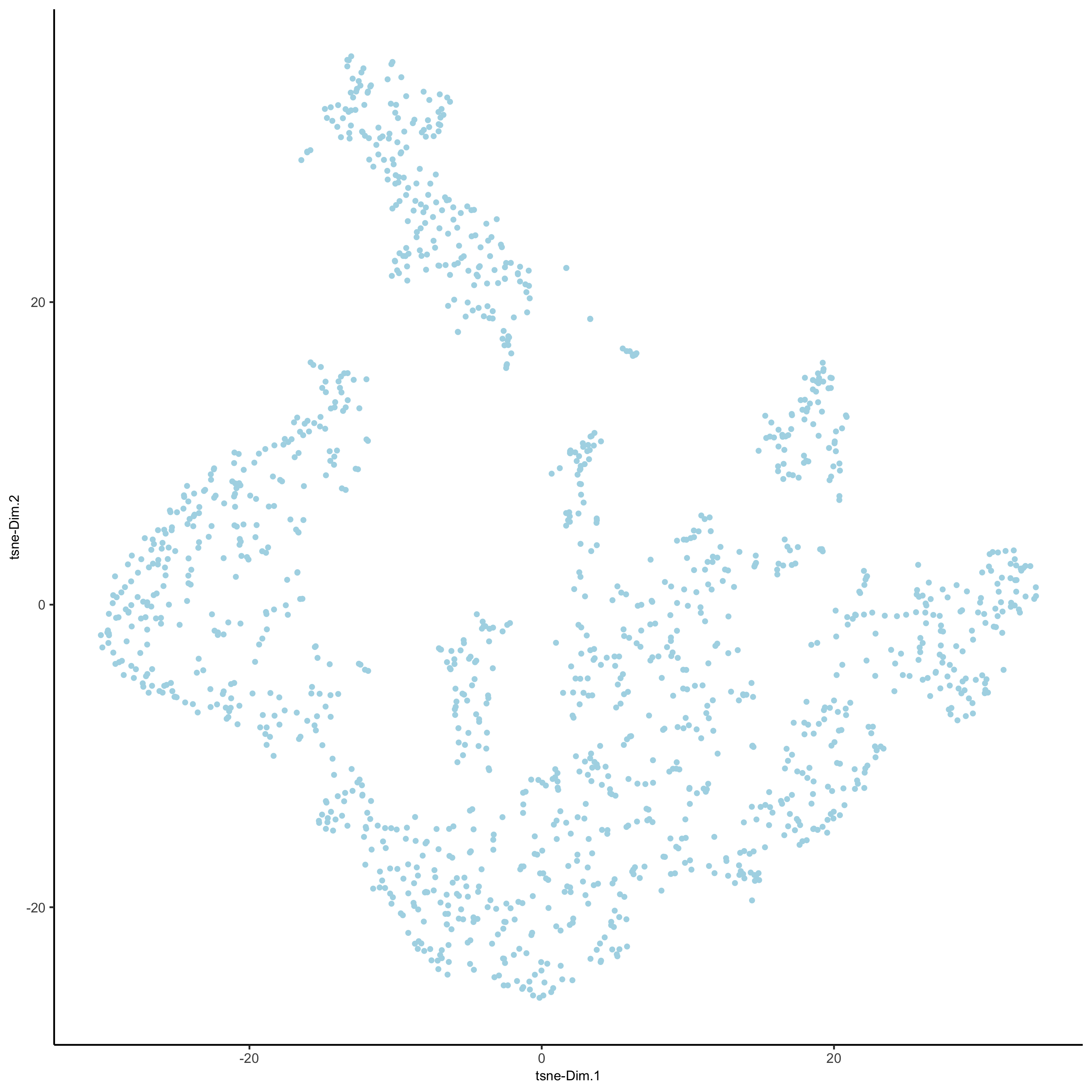## 4. Clustering¶

```## sNN network (default)
visium_kidney <- createNearestNetwork(gobject = visium_kidney, dimensions_to_use = 1:10, k = 15)
## Leiden clustering
visium_kidney <- doLeidenCluster(gobject = visium_kidney, resolution = 0.4, n_iterations = 1000)
plotUMAP(gobject = visium_kidney,
cell_color = 'leiden_clus', show_NN_network = T, point_size = 2.5,
save_param = list(save_name = '4_a_UMAP_leiden'))
```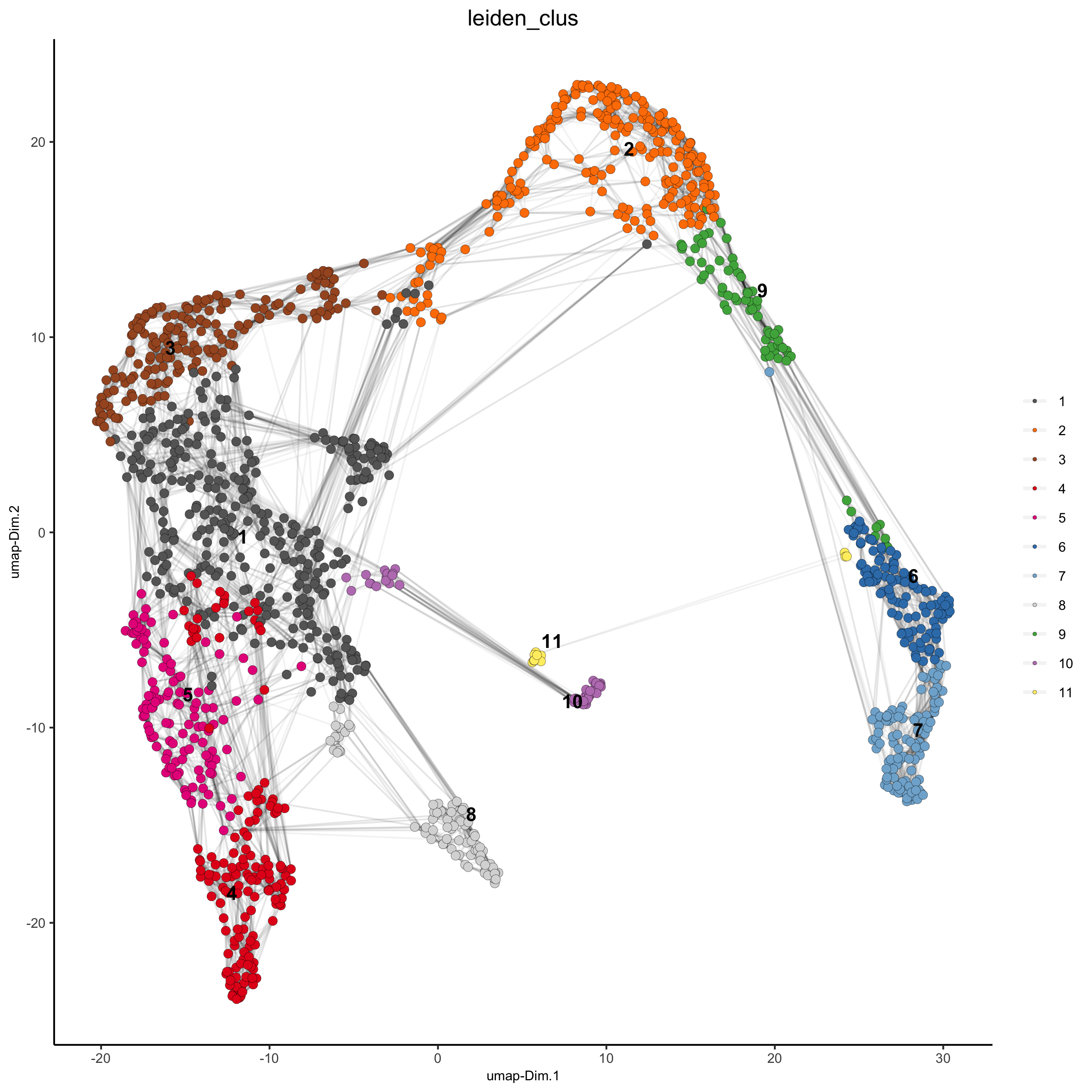## 5. Co-Visualize¶

```# expression and spatial
spatDimPlot(gobject = visium_kidney, cell_color = 'leiden_clus',
dim_point_size = 2, spat_point_size = 2.5,
save_param = list(save_name = '5_a_covis_leiden'))
```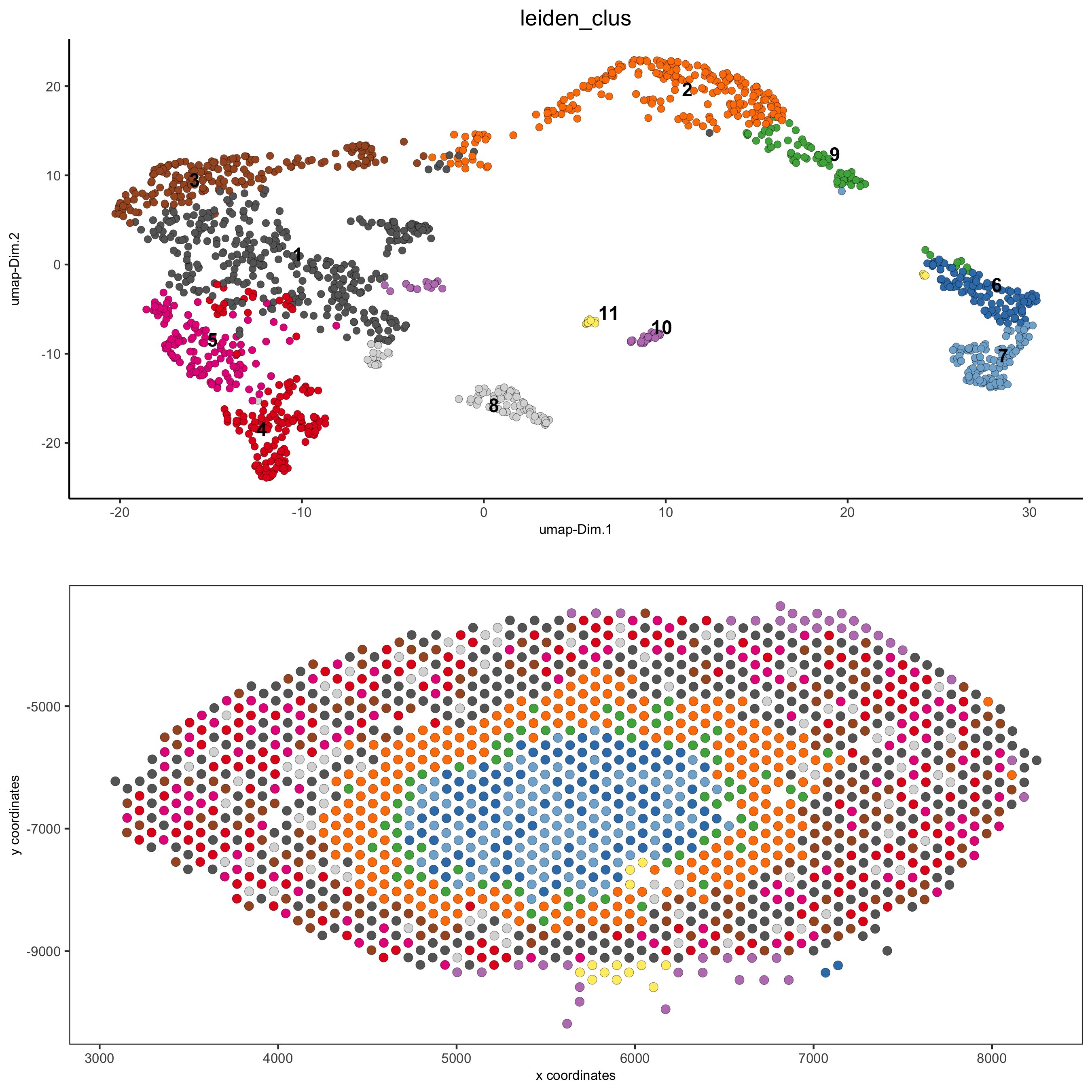```spatDimPlot(gobject = visium_kidney, cell_color = 'nr_genes', color_as_factor = F,
dim_point_size = 2, spat_point_size = 2.5,
save_param = list(save_name = '5_b_nr_genes'))
```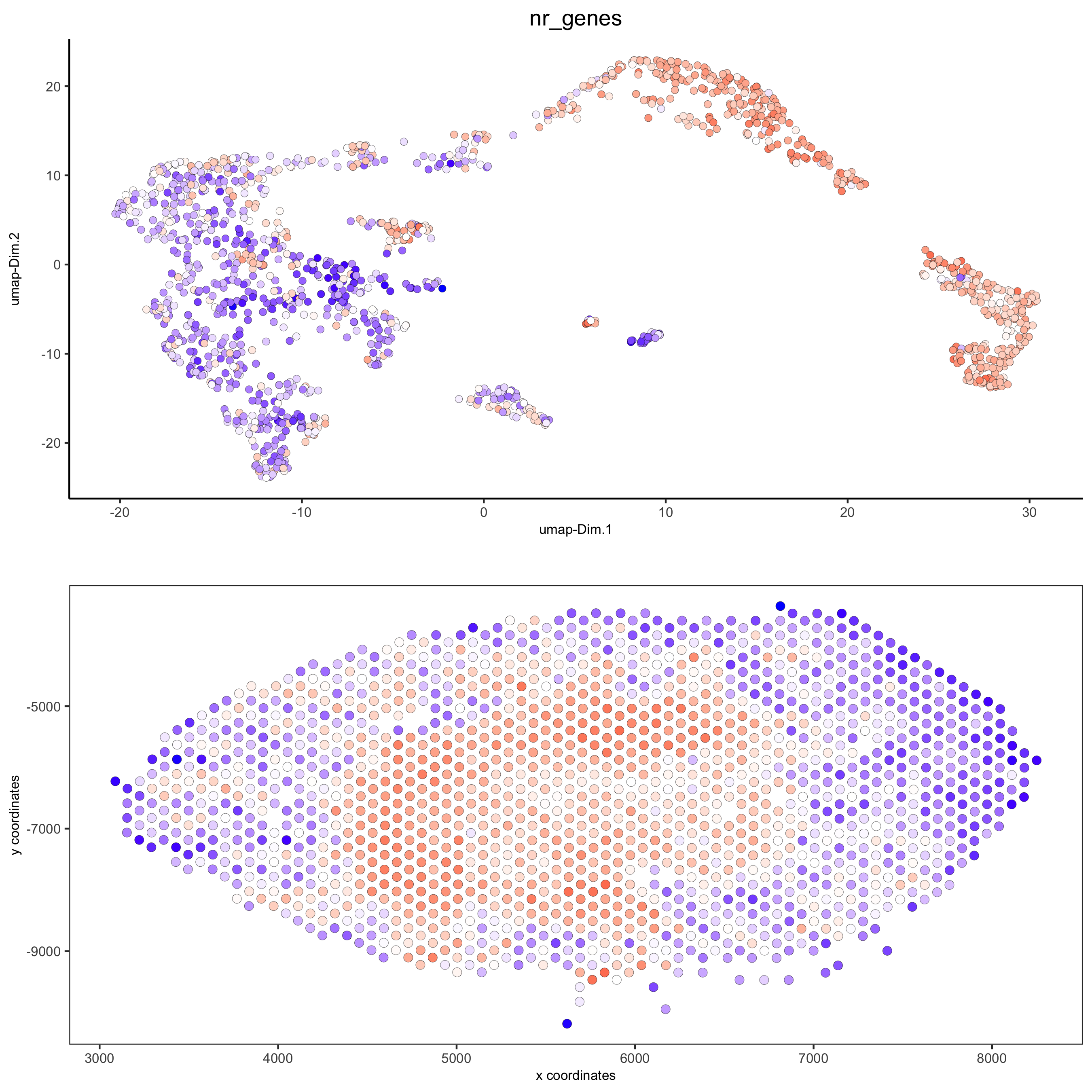## 6. Cell-Type Marker Gene Detection¶

### 6.1 Gini¶

```gini_markers_subclusters = findMarkers_one_vs_all(gobject = visium_kidney,
method = 'gini',
expression_values = 'normalized',
cluster_column = 'leiden_clus',
min_genes = 20,
min_expr_gini_score = 0.5,
min_det_gini_score = 0.5)
topgenes_gini = gini_markers_subclusters[, head(.SD, 2), by = 'cluster']\$genes

# violinplot
violinPlot(visium_kidney, genes = unique(topgenes_gini), cluster_column = 'leiden_clus',
strip_text = 8, strip_position = 'right',
save_param = c(save_name = '6_a_violinplot_gini', base_width = 5, base_height = 10))
```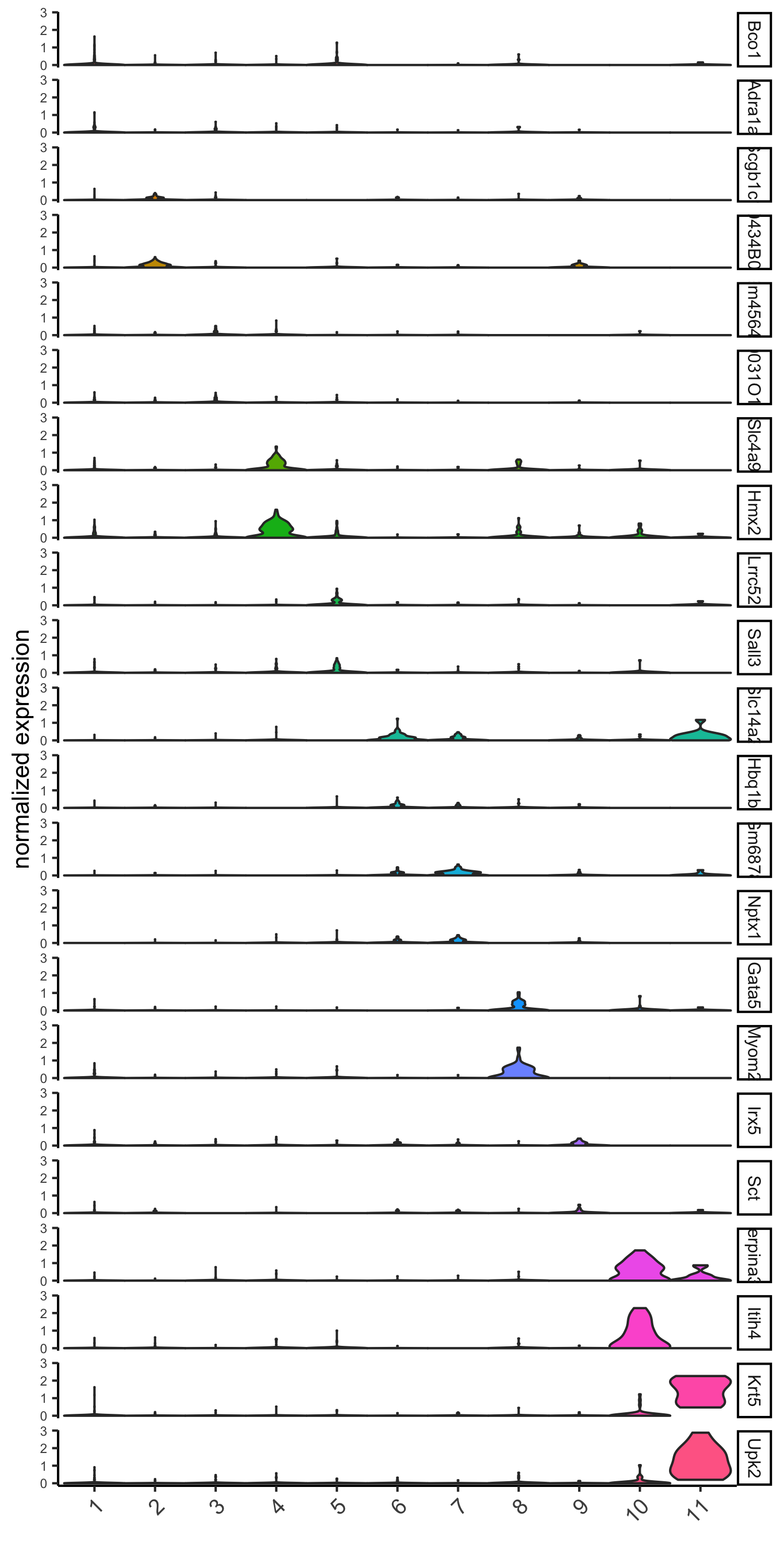```# cluster heatmap
x_text_size = 10, y_text_size = 10,
save_param = c(save_name = '6_b_metaheatmap_gini'))
```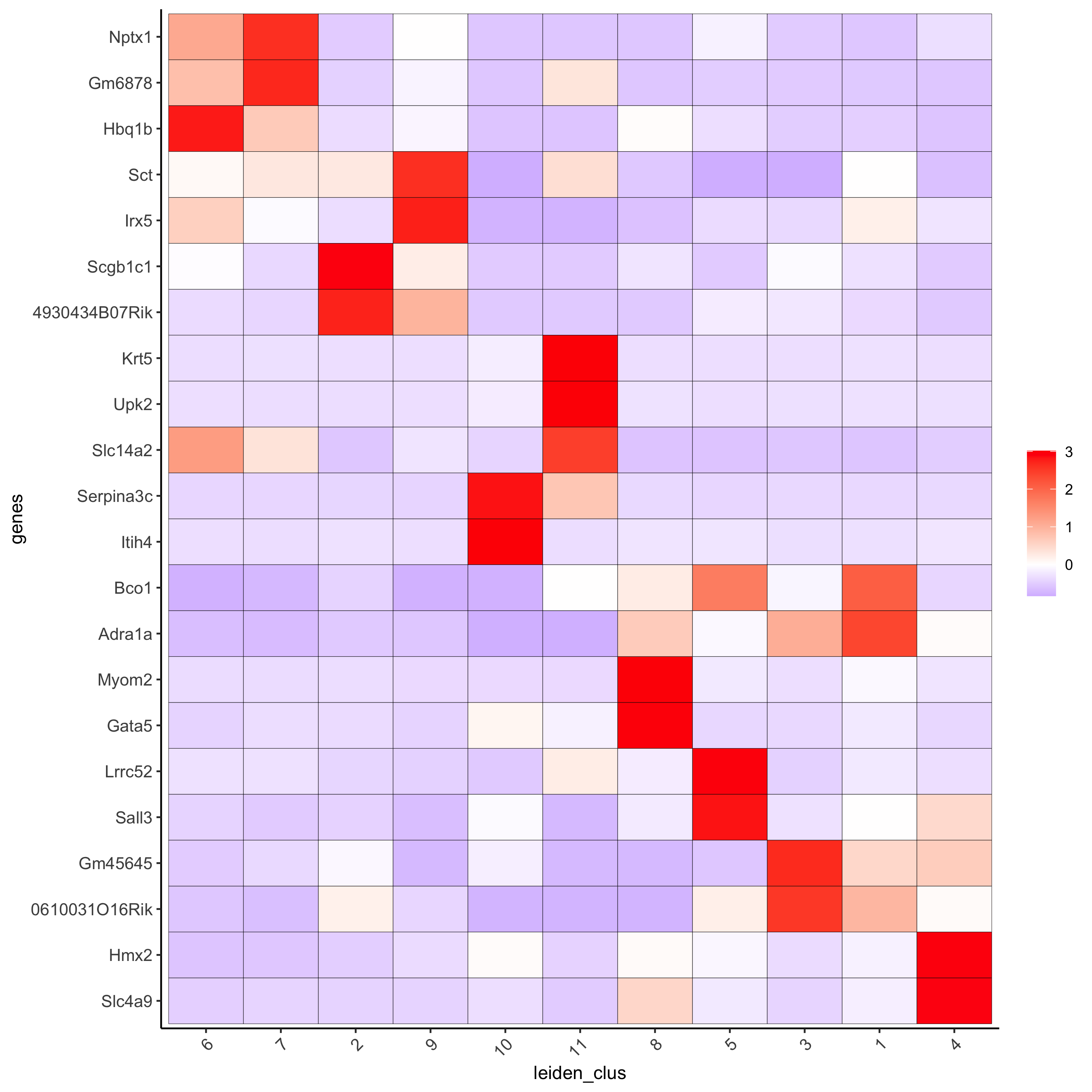```# umap plots
dimGenePlot2D(visium_kidney, expression_values = 'scaled',
genes = gini_markers_subclusters[, head(.SD, 1), by = 'cluster']\$genes,
cow_n_col = 3, point_size = 1,
save_param = c(save_name = '6_c_gini_umap', base_width = 8, base_height = 5))
```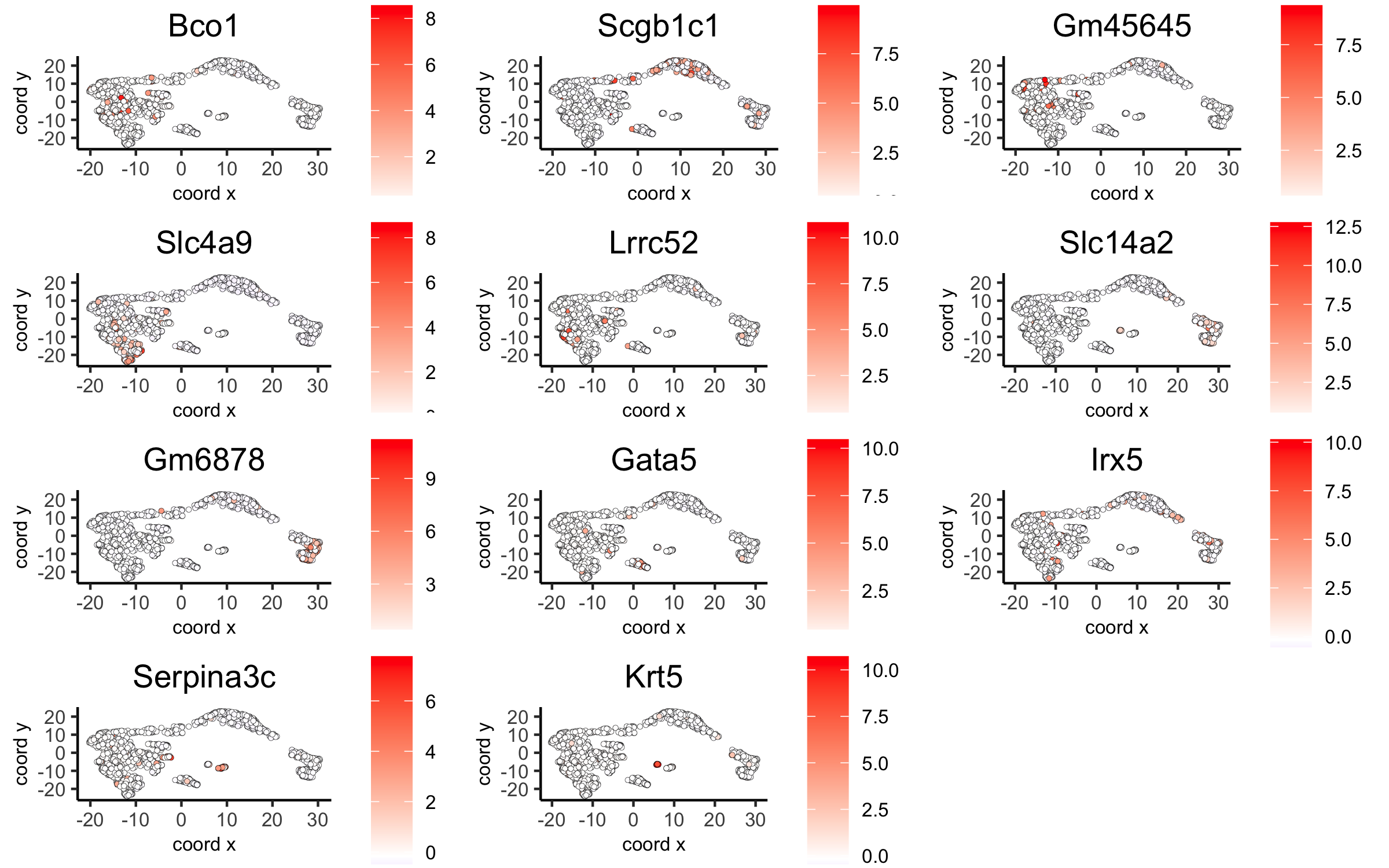### 6.2 Scran¶

```scran_markers_subclusters = findMarkers_one_vs_all(gobject = visium_kidney,
method = 'scran',
expression_values = 'normalized',
cluster_column = 'leiden_clus')
topgenes_scran = scran_markers_subclusters[, head(.SD, 2), by = 'cluster']\$genes

# violinplot
violinPlot(visium_kidney, genes = unique(topgenes_scran), cluster_column = 'leiden_clus',
strip_text = 10, strip_position = 'right',
save_param = c(save_name = '6_d_violinplot_scran', base_width = 5))
```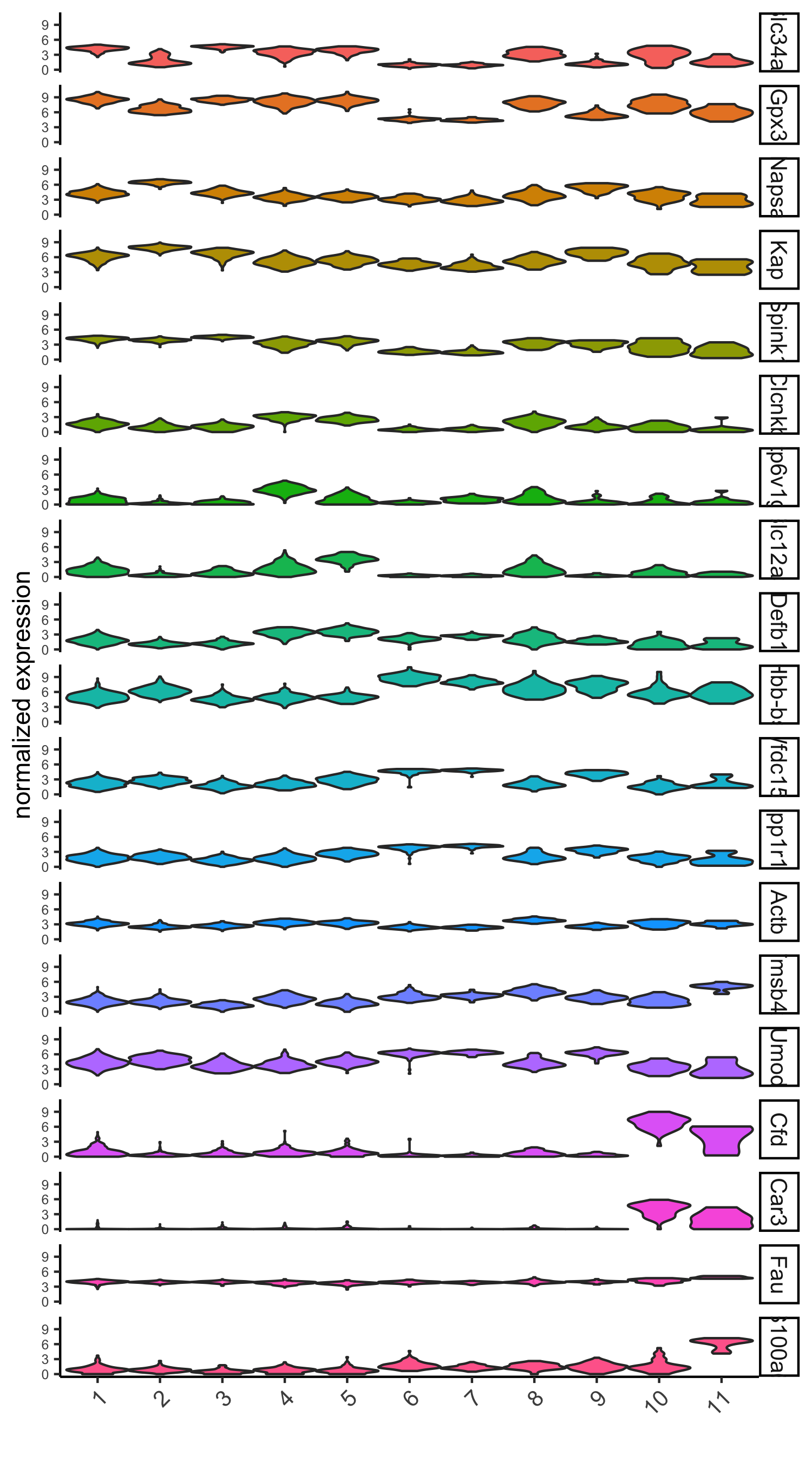```# cluster heatmap
save_param = c(save_name = '6_e_metaheatmap_scran'))
```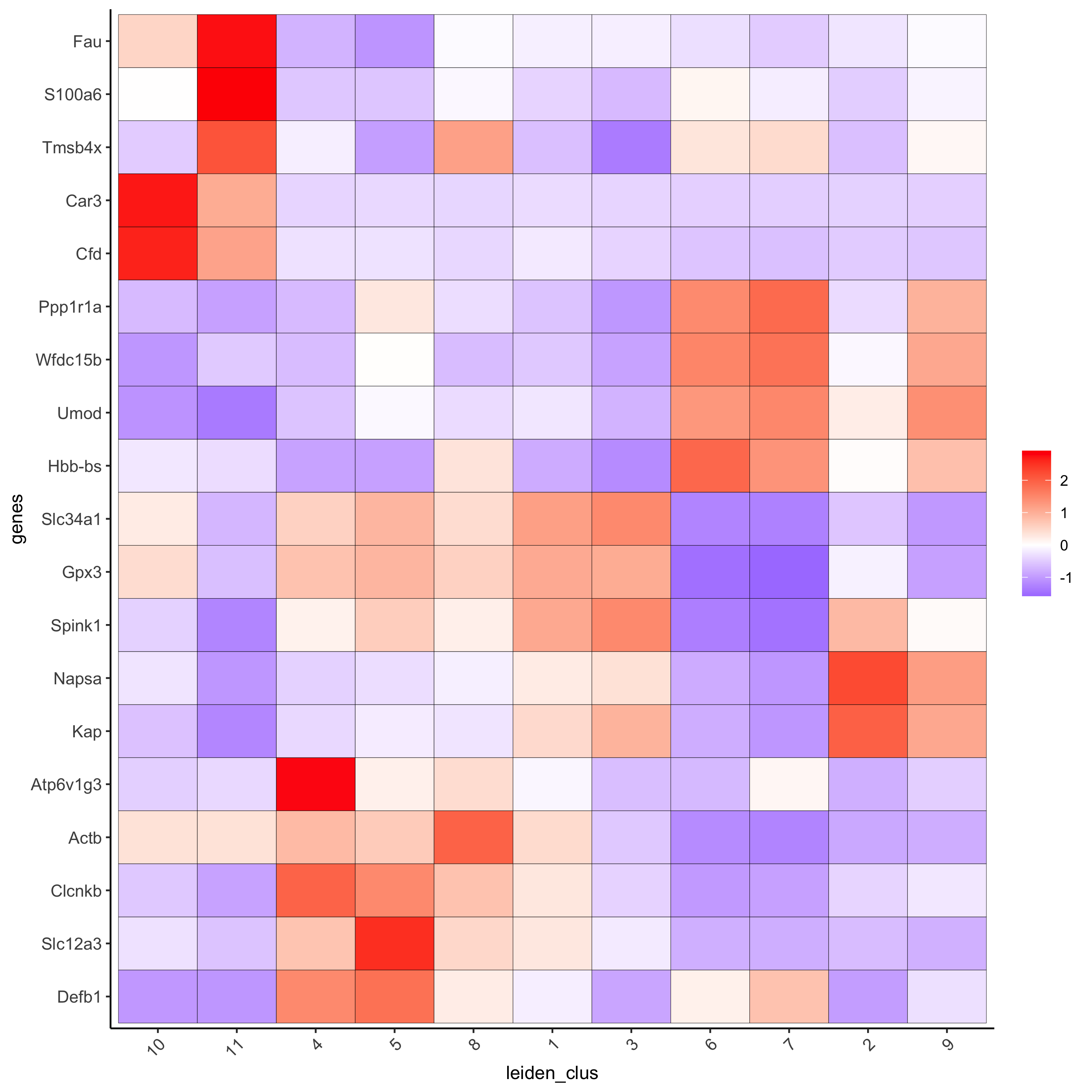```# umap plots
dimGenePlot(visium_kidney, expression_values = 'scaled',
genes = scran_markers_subclusters[, head(.SD, 1), by = 'cluster']\$genes,
cow_n_col = 3, point_size = 1,
save_param = c(save_name = '6_f_scran_umap', base_width = 8, base_height = 5))
```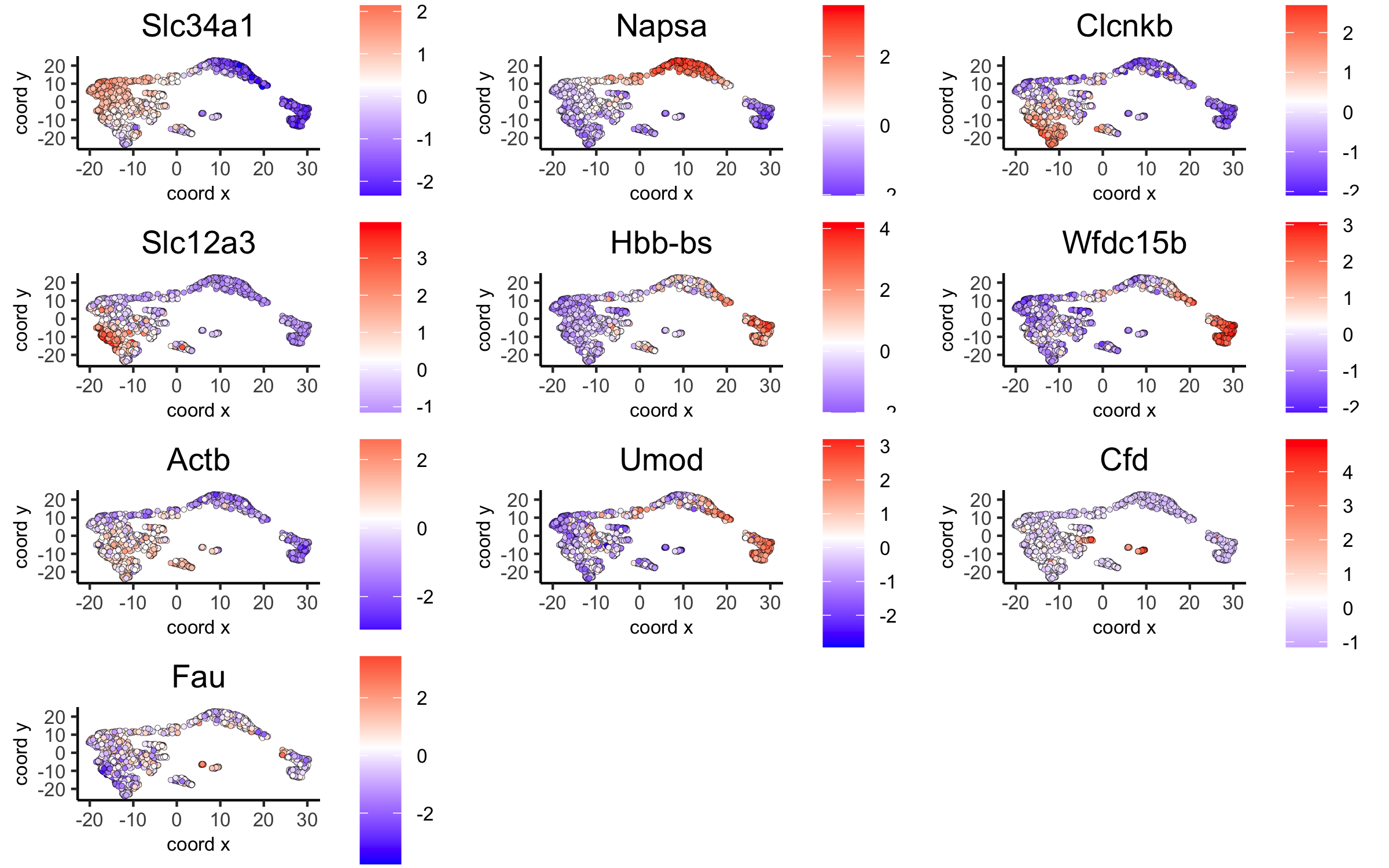## 7. Cell-Type Annotation¶

Visium spatial transcriptomics does not provide single-cell resolution, making cell type annotation a harder problem. Giotto provides 3 ways to calculate enrichment of specific cell-type signature gene list:

• PAGE

• RANK

• Hypergeometric Test

See the Mouse Visium Brain Dataset for an example.

## 8. Spatial Grid¶

```visium_kidney <- createSpatialGrid(gobject = visium_kidney,
sdimx_stepsize = 400,
sdimy_stepsize = 400,
spatPlot(visium_kidney, cell_color = 'leiden_clus', show_grid = T,
grid_color = 'red', spatial_grid_name = 'spatial_grid',
save_param = c(save_name = '8_grid'))
```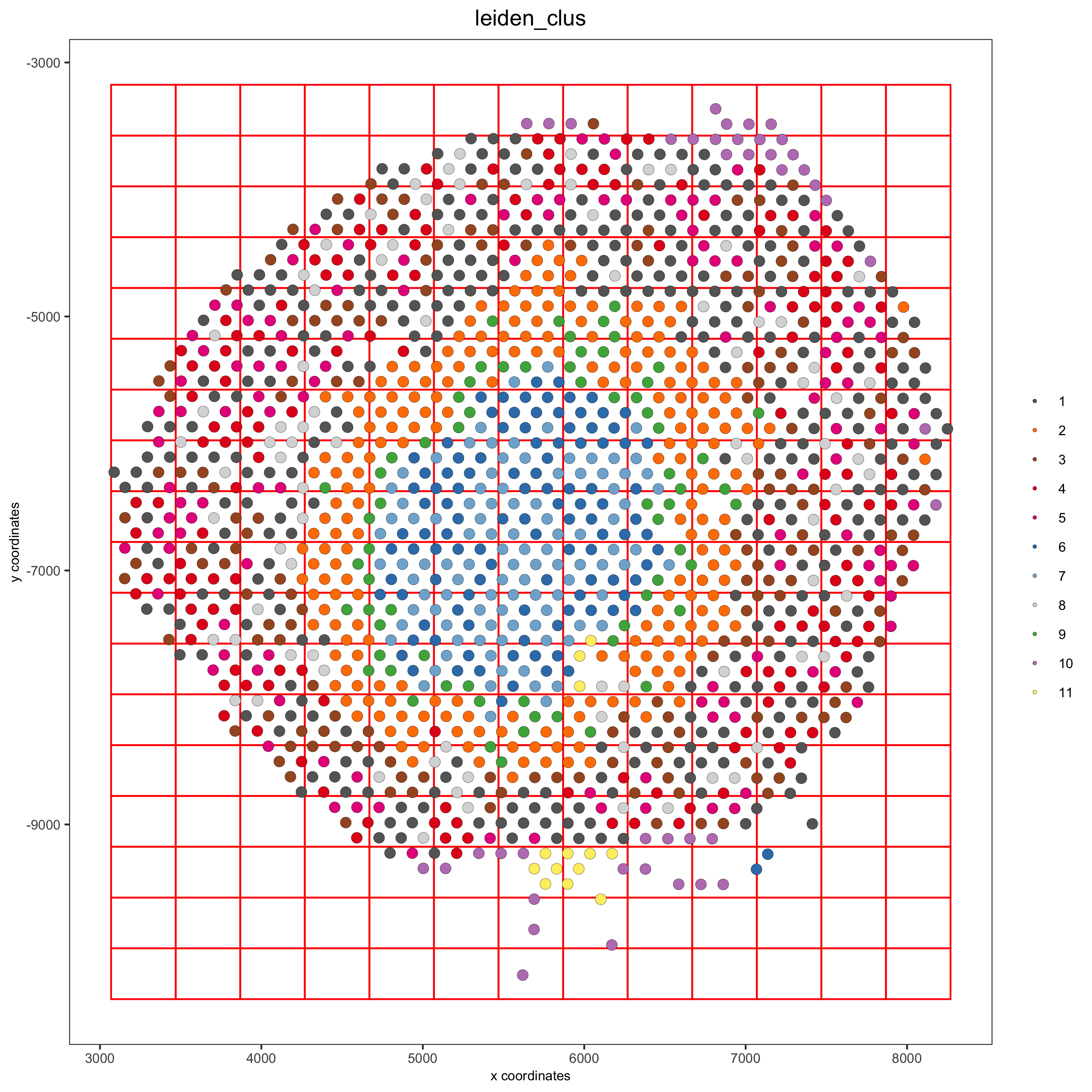## 9. Spatial Network¶

```## delaunay network: stats + creation
plotStatDelaunayNetwork(gobject = visium_kidney, maximum_distance = 400,
save_param = c(save_name = '9_a_delaunay_network'))
```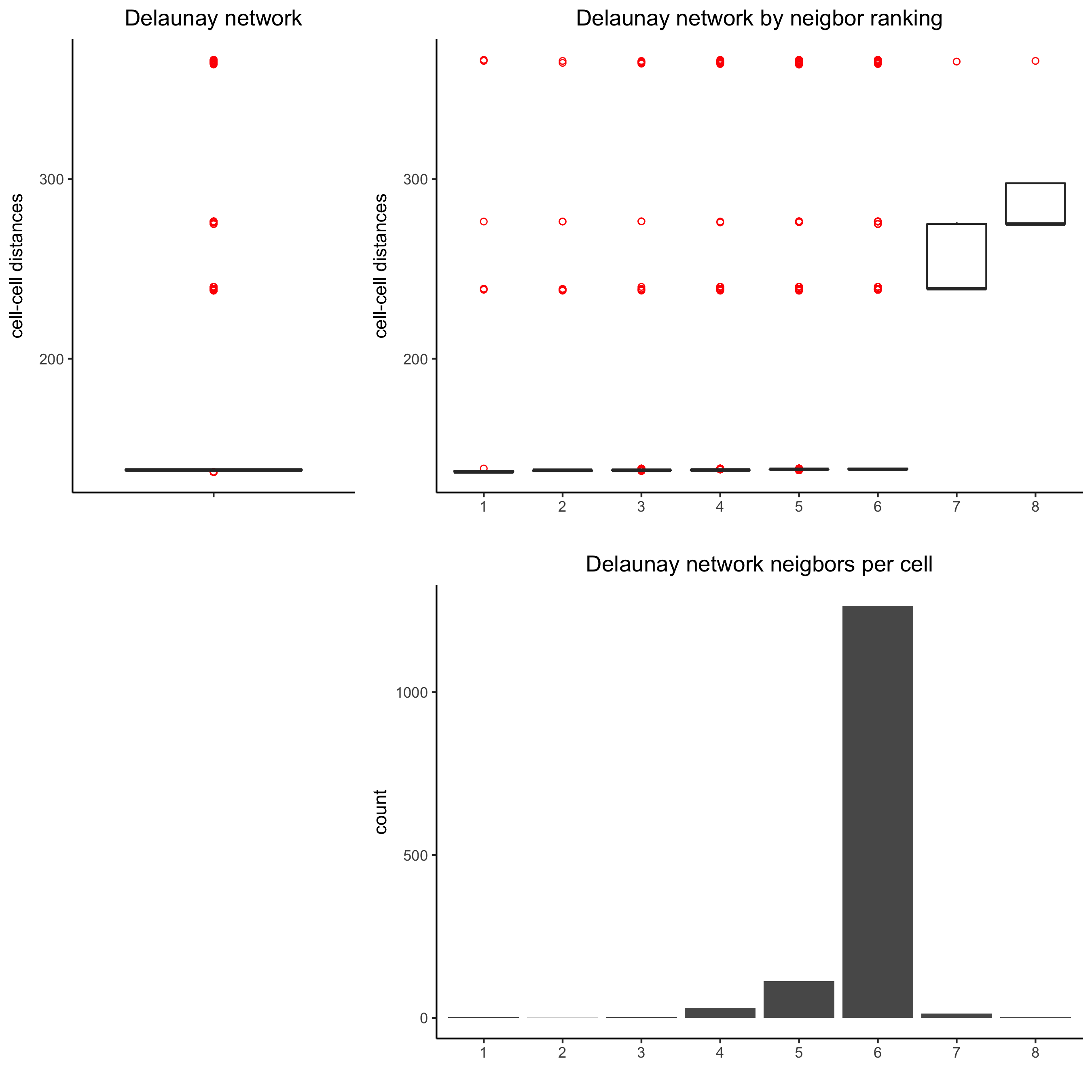```visium_kidney = createSpatialNetwork(gobject = visium_kidney, minimum_k = 0)
showNetworks(visium_kidney)
spatPlot(gobject = visium_kidney, show_network = T,
network_color = 'blue', spatial_network_name = 'Delaunay_network',
save_param = c(save_name = '9_b_delaunay_network'))
```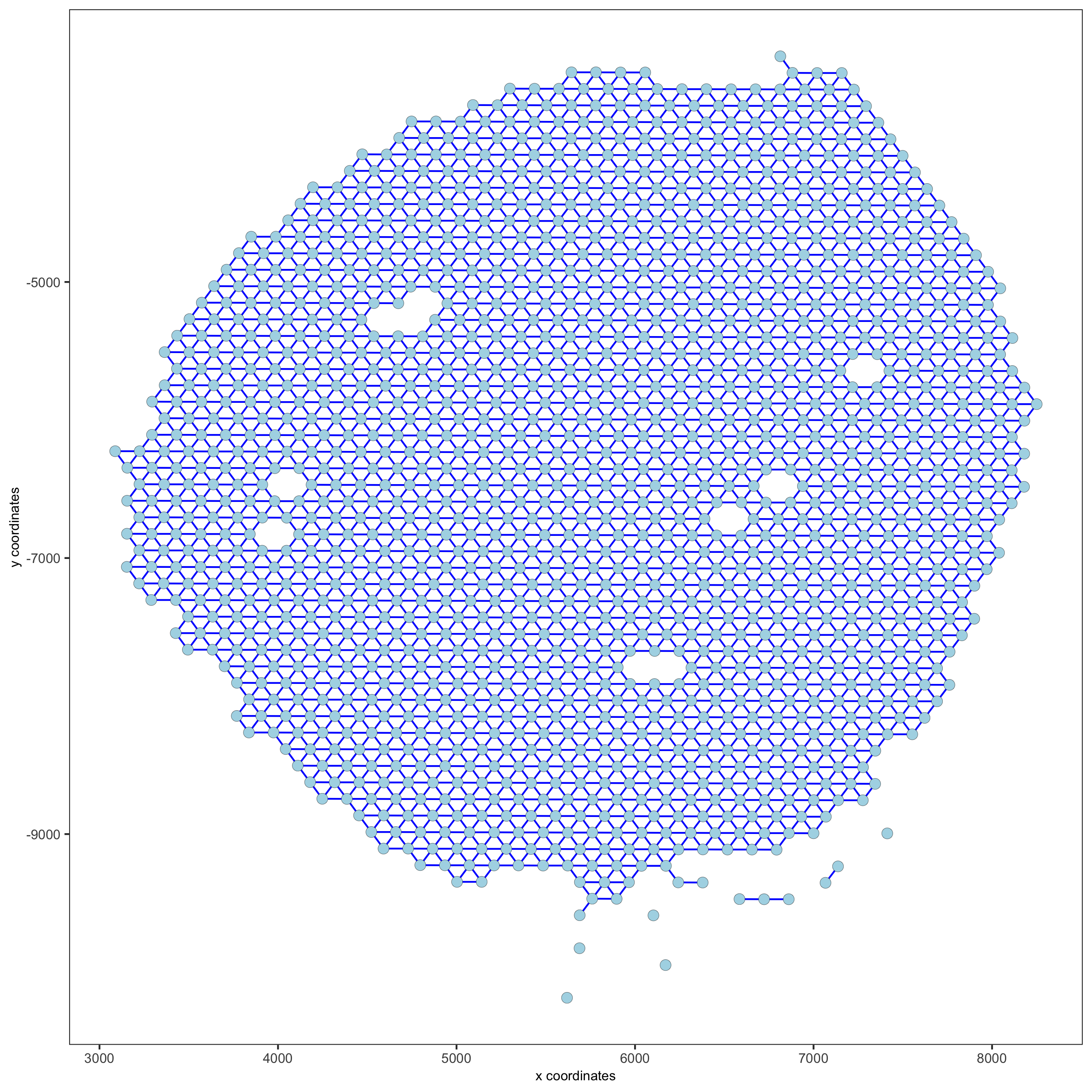## 10. Spatial Genes and Co-Expression Patterns¶

### 10.1 Spatial Genes¶

```## kmeans binarization
kmtest = binSpect(visium_kidney)
spatGenePlot(visium_kidney, expression_values = 'scaled',
genes = kmtest\$genes[1:6], cow_n_col = 2, point_size = 1.5,
save_param = c(save_name = '10_a_spatial_genes_km'))
```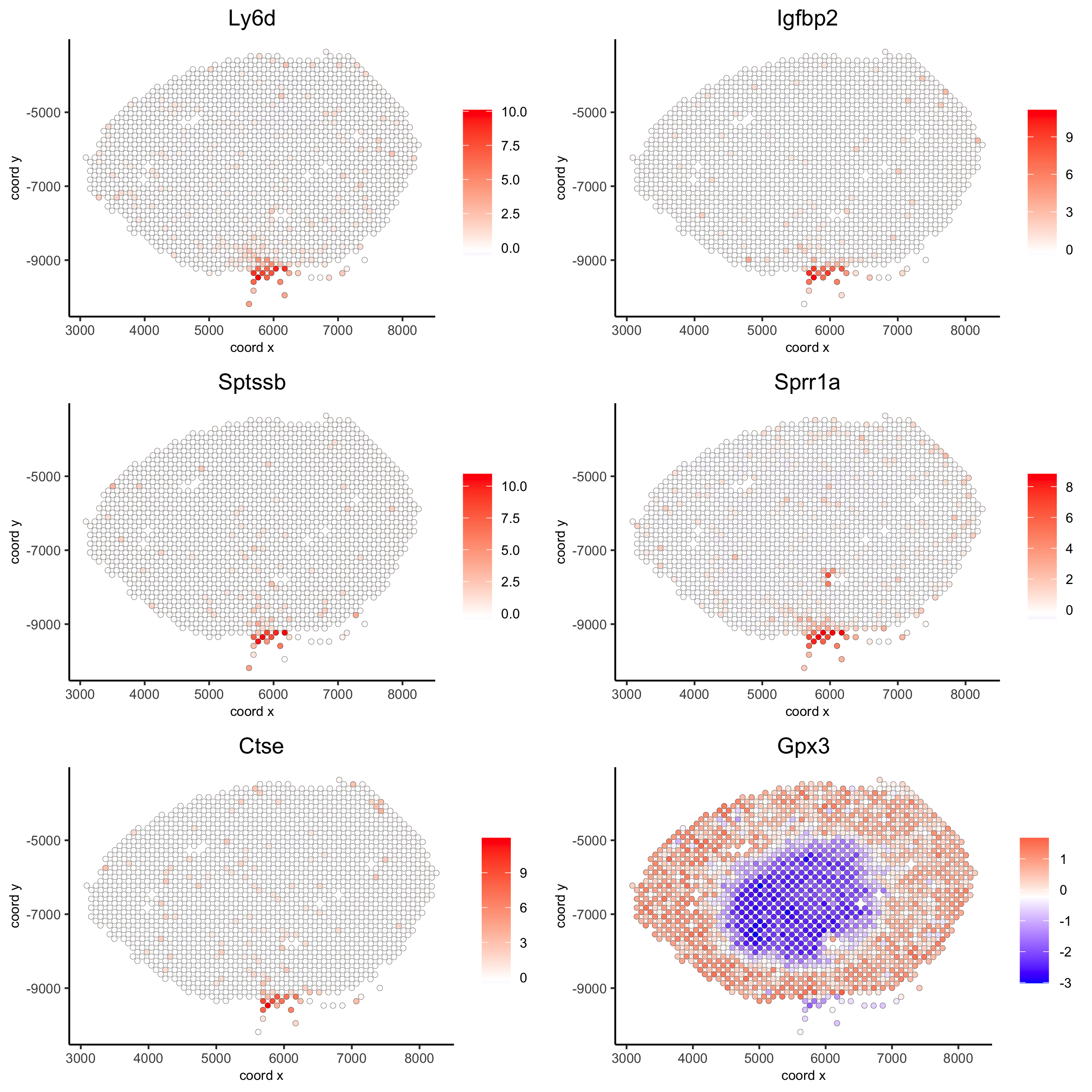```## rank binarization
ranktest = binSpect(visium_kidney, bin_method = 'rank')
spatGenePlot(visium_kidney, expression_values = 'scaled',
genes = ranktest\$genes[1:6], cow_n_col = 2, point_size = 1.5,
save_param = c(save_name = '10_b_spatial_genes_rank'))
```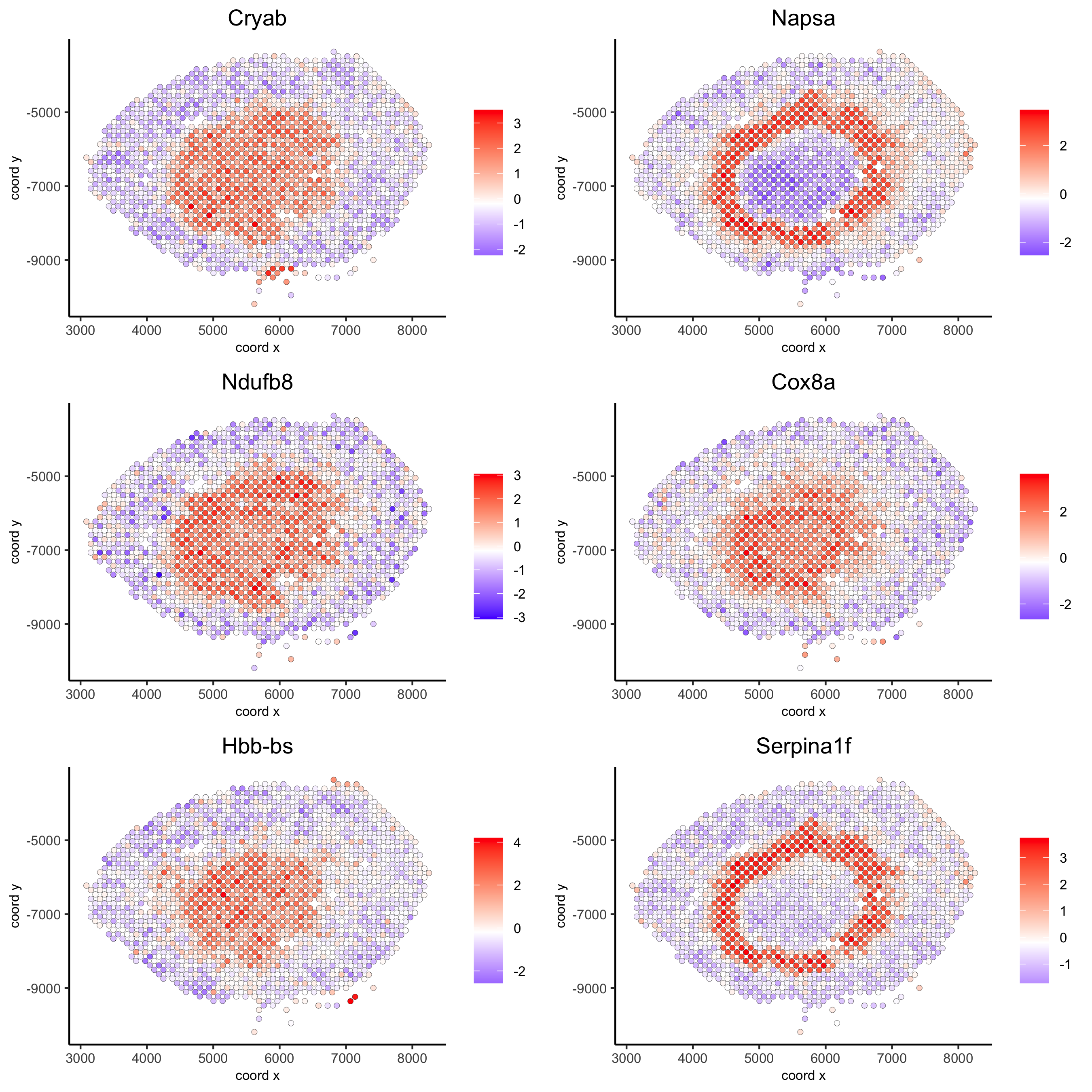### 10.2 Spatial Co-Expression Patterns¶

```## spatially correlated genes ##
ext_spatial_genes = kmtest[1:500]\$genes

# 1. calculate gene spatial correlation and single-cell correlation
# create spatial correlation object
spat_cor_netw_DT = detectSpatialCorGenes(visium_kidney,
method = 'network',
spatial_network_name = 'Delaunay_network',
subset_genes = ext_spatial_genes)

# 2. identify most similar spatially correlated genes for one gene
Napsa_top10_genes = showSpatialCorGenes(spat_cor_netw_DT, genes = 'Napsa', show_top_genes = 10)
spatGenePlot(visium_kidney, expression_values = 'scaled',
genes = c('Napsa', 'Kap', 'Defb29', 'Prdx1'), point_size = 3,
save_param = c(save_name = '10_d_Napsa_correlated_genes'))
```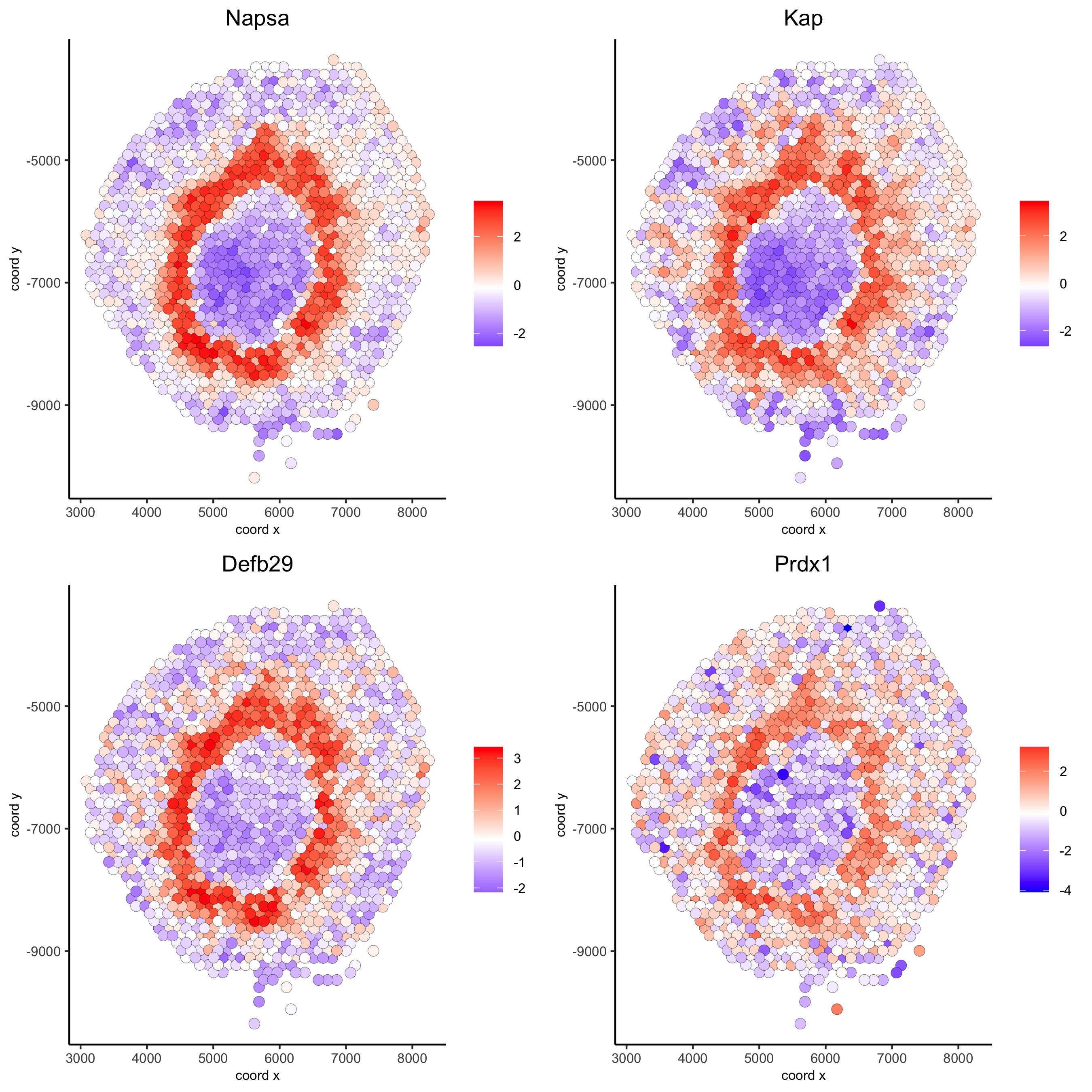```# 3. cluster correlated genes & visualize
spat_cor_netw_DT = clusterSpatialCorGenes(spat_cor_netw_DT, name = 'spat_netw_clus', k = 8)

heatmSpatialCorGenes(visium_kidney, spatCorObject = spat_cor_netw_DT, use_clus_name = 'spat_netw_clus',
save_param = c(save_name = '10_e_heatmap_correlated_genes', save_format = 'pdf',
base_height = 6, base_width = 8, units = 'cm'),
heatmap_legend_param = list(title = NULL))
``````# 4. rank spatial correlated clusters and show genes for selected clusters
netw_ranks = rankSpatialCorGroups(visium_kidney, spatCorObject = spat_cor_netw_DT, use_clus_name = 'spat_netw_clus',
save_param = c(save_name = '10_f_rank_correlated_groups',
base_height = 3, base_width = 5))
```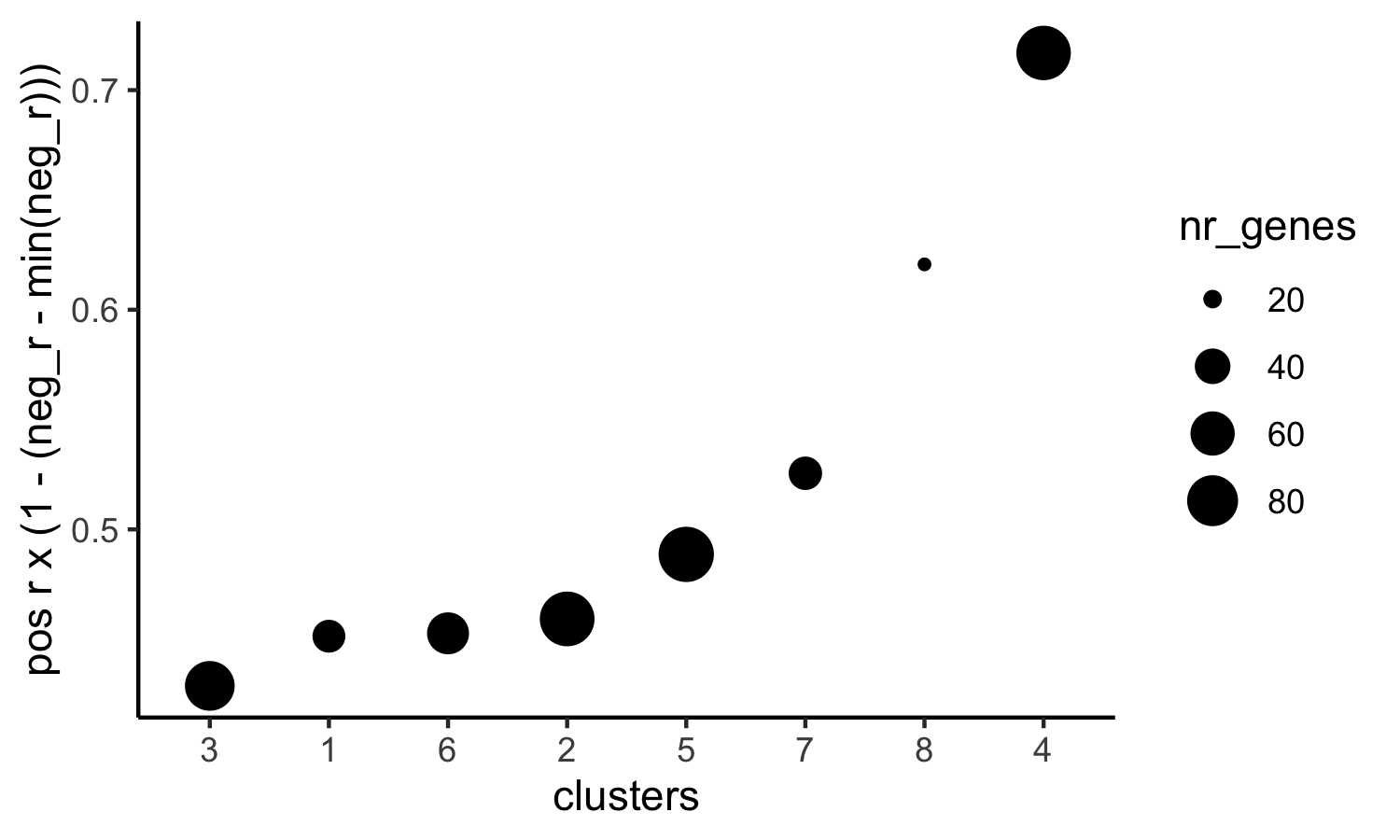```top_netw_spat_cluster = showSpatialCorGenes(spat_cor_netw_DT, use_clus_name = 'spat_netw_clus',
selected_clusters = 6, show_top_genes = 1)

# 5. create metagene enrichment score for clusters
cluster_genes_DT = showSpatialCorGenes(spat_cor_netw_DT, use_clus_name = 'spat_netw_clus', show_top_genes = 1)
cluster_genes = cluster_genes_DT\$clus; names(cluster_genes) = cluster_genes_DT\$gene_ID

visium_kidney = createMetagenes(visium_kidney, gene_clusters = cluster_genes, name = 'cluster_metagene')

spatCellPlot(visium_kidney,
spat_enr_names = 'cluster_metagene',
cell_annotation_values = netw_ranks\$clusters,
point_size = 1.5, cow_n_col = 4,
save_param = c(save_name = '10_g_spat_enrichment_score_plots',
base_width = 13, base_height = 6))
```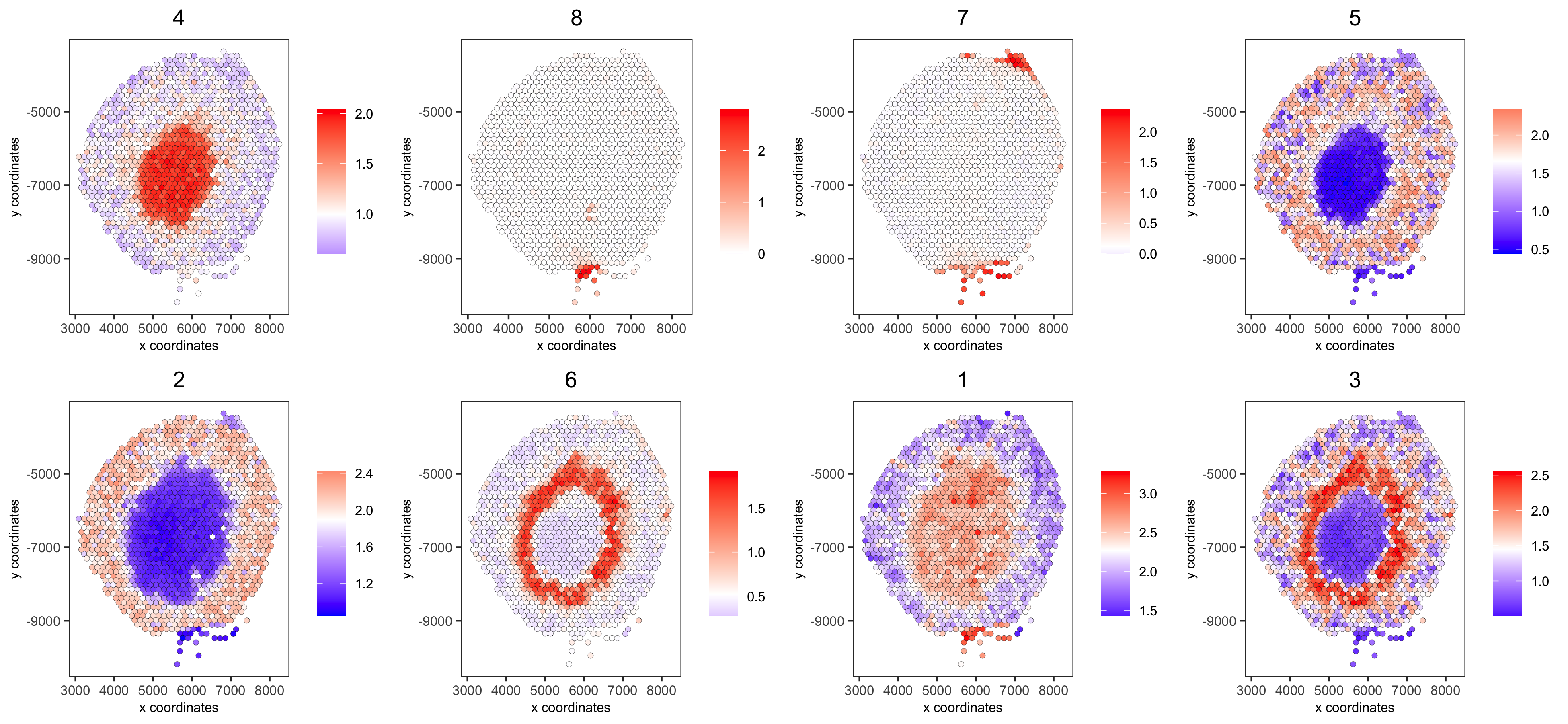```# example for gene per cluster
top_netw_spat_cluster = showSpatialCorGenes(spat_cor_netw_DT, use_clus_name = 'spat_netw_clus',
selected_clusters = 1:8, show_top_genes = 1)
first_genes = top_netw_spat_cluster[, head(.SD, 1), by = clus]\$gene_ID
cluster_names = top_netw_spat_cluster[, head(.SD, 1), by = clus]\$clus
names(first_genes) = cluster_names
first_genes = first_genes[as.character(netw_ranks\$clusters)]

spatGenePlot(visium_kidney, genes = first_genes, expression_values = 'scaled', cow_n_col = 4, midpoint = 0, point_size = 2,
save_param = c(save_name = '10_h_spat_enrichment_score_plots_genes',
base_width = 11, base_height = 6))
```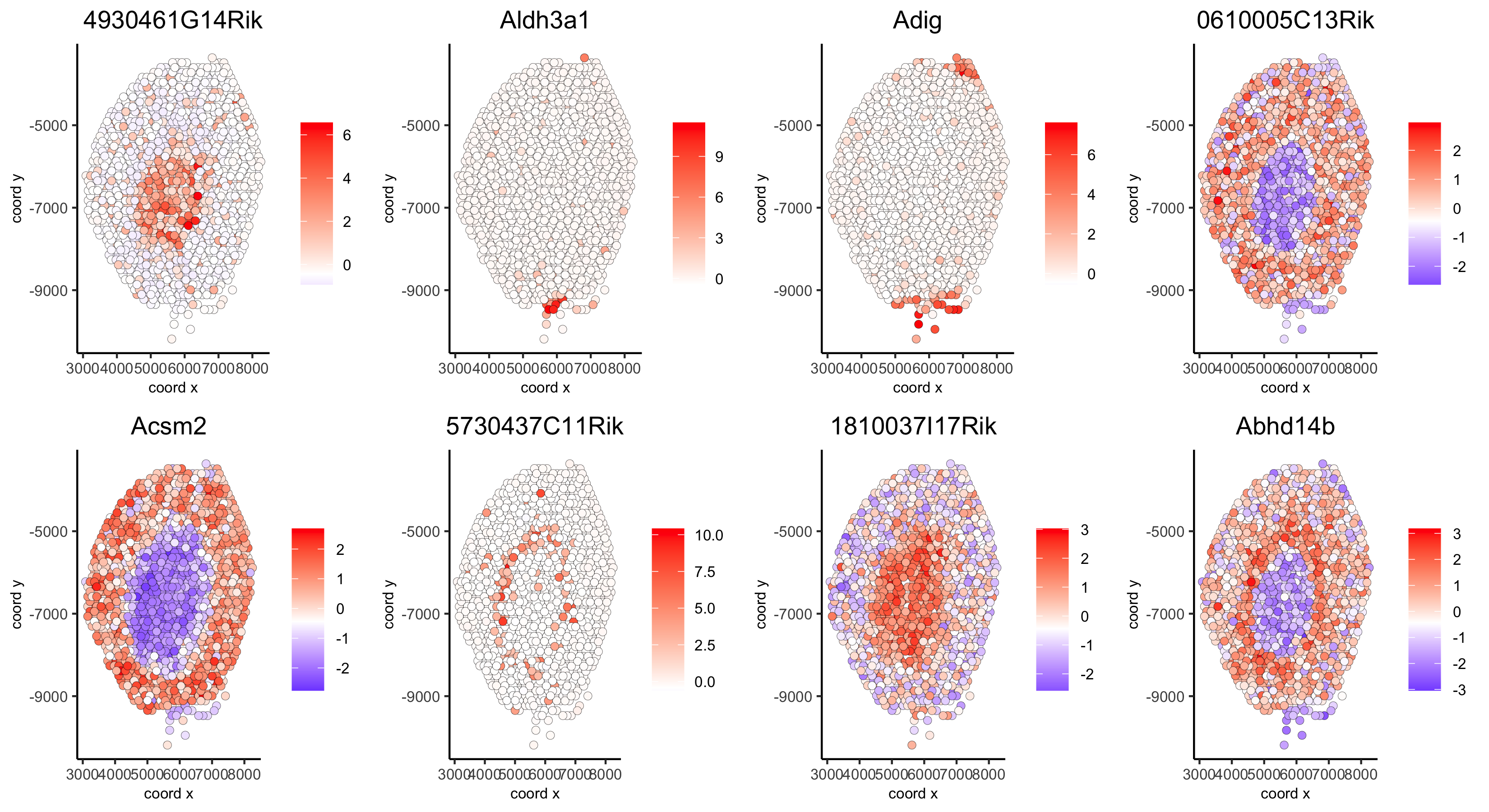## 11. HMRF Domains¶

```# HMRF requires a fully connected network!
visium_kidney = createSpatialNetwork(gobject = visium_kidney, minimum_k = 2, name = 'Delaunay_full')

# spatial genes
my_spatial_genes <- kmtest[1:100]\$genes

# do HMRF with different betas
hmrf_folder = paste0(results_folder,'/','11_HMRF/')
if(!file.exists(hmrf_folder)) dir.create(hmrf_folder, recursive = T)

HMRF_spatial_genes = doHMRF(gobject = visium_kidney, expression_values = 'scaled',
spatial_network_name = 'Delaunay_full',
spatial_genes = my_spatial_genes,
k = 5,
betas = c(0, 1, 6),
output_folder = paste0(hmrf_folder, '/', 'Spatial_genes/SG_topgenes_k5_scaled'))

## view results of HMRF
for(i in seq(0, 5, by = 1)) {
viewHMRFresults2D(gobject = visium_kidney,
HMRFoutput = HMRF_spatial_genes,
k = 5, betas_to_view = i,
point_size = 2)
}
```

Alternative Way to View Results

```#results = writeHMRFresults(gobject = ST_test,
#                           HMRFoutput = HMRF_spatial_genes,
#                           k = 5, betas_to_view = seq(0, 25, by = 5))

## add HMRF of interest to giotto object
HMRFoutput = HMRF_spatial_genes,
k = 5, betas_to_add = c(0, 2),
hmrf_name = 'HMRF')

## visualize
spatPlot(gobject = visium_kidney, cell_color = 'HMRF_k5_b.0', point_size = 5,
save_param = c(save_name = '11_a_HMRF_k5_b.0'))
```
```spatPlot(gobject = visium_kidney, cell_color = 'HMRF_k5_b.2', point_size = 5,
save_param = c(save_name = '11_b_HMRF_k5_b.2'))
```

## Export and Create Giotto Viewer¶

```# check which annotations are available

# select annotations, reductions and expression values to view in Giotto Viewer
viewer_folder = paste0(results_folder, '/', 'mouse_visium_kidney_viewer')

exportGiottoViewer(gobject = visium_kidney,
output_directory = viewer_folder,
spat_enr_names = 'PAGE',
factor_annotations = c('in_tissue',
'leiden_clus'),
numeric_annotations = c('nr_genes',
'clus_25'),
dim_reductions = c('tsne', 'umap'),
dim_reduction_names = c('tsne', 'umap'),
expression_values = 'scaled',
expression_rounding = 2,
overwrite_dir = T)
```